# Probabilistic Inference of Simulation Parameters via Parallel Differentiable Simulation

## ICRA 2022 (Under Review)

1University of Southern California

2NVIDIA

3University of Sydney

4Amazon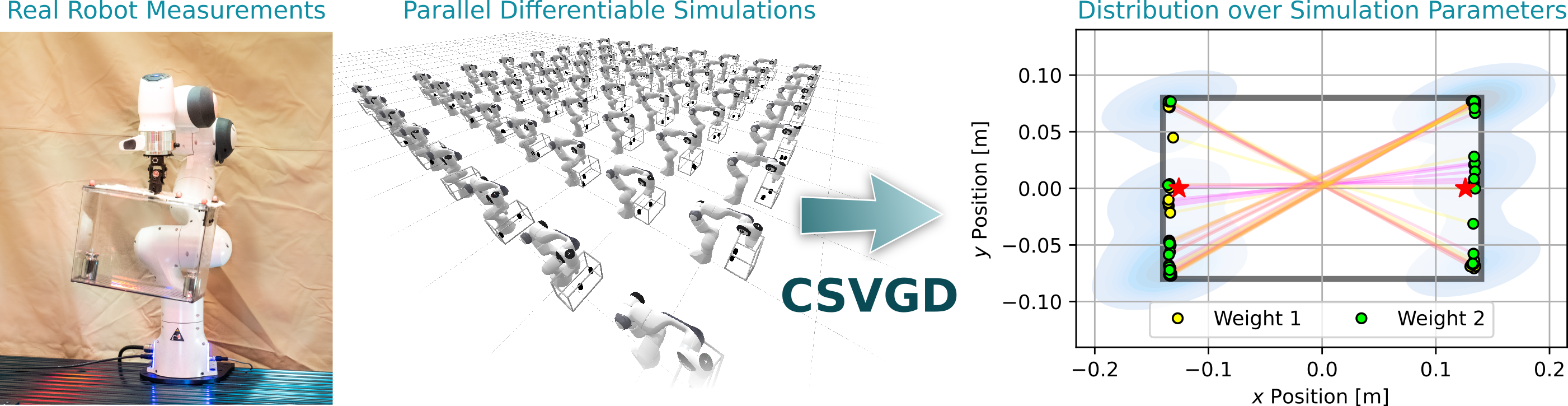We reduce the reality gap in robotics simulators by introducing a Bayesian inference approach named Constrained Stein Variational Gradient Descent (CSVGD). Through a multiple-shooting likelihood model for trajectories, and by leveraging parallel differentiable simulators, CSVGD can infer complex, non-parametric posterior distributions over simulation parameters for highly nonlinear systems.

## Abstract

To accurately reproduce measurements from the real world, simulators need to have an adequate model of the physical system and require the parameters of the model be identified.

We address the latter problem of estimating parameters through a Bayesian inference approach that approximates a posterior distribution over simulation parameters given real sensor measurements. By extending the commonly used Gaussian likelihood model for trajectories via the multiple-shooting formulation, our chosen particle-based inference algorithm Stein Variational Gradient Descent is able to identify highly nonlinear, underactuated systems. We leverage GPU code generation and differentiable simulation to evaluate the likelihood and its gradient for many particles in parallel.

Our algorithm infers non-parametric distributions over simulation parameters more accurately than comparable baselines and handles constraints over parameters efficiently through gradient-based optimization. We evaluate estimation performance on several physical experiments. On an underactuated mechanism where a 7-DOF robot arm excites an object with an unknown mass configuration, we demonstrate how our inference technique can identify symmetries between the parameters and provide highly accurate predictions.

## Appendix

The following sections provide additional technical details that supplement our paper. Please consult our paper for the definition of the symbols used in the following sections.

$\gdef\paramdim{M} \gdef\params{\theta} \gdef\particles{\Theta} \gdef\numparticles{N} \gdef\statedim{D} \gdef\statevec{\mathbf{s}} \gdef\controlvec{\mathbf{u}} \gdef\observationdim{N} \gdef\observationvec{\mathbf{x}} \gdef\timedim{T} \gdef\trajectory{\mathcal{X}} \gdef\trajectoryset{D_\trajectory} \gdef\trajectorysetsub{D_{\trajectory,#1}} \gdef\particleset{\xi} \gdef\simu{^{\text{sim}}} \gdef\real{^{\text{real}}} \gdef\fsim{f_{\text{sim}}} \gdef\fobs{f_{\text{obs}}} \gdef\fstep{f_{\text{step}}} \gdef\pobs{p_{\text{obs}}} \gdef\xp{\params^\prime} \gdef\kxx{k(\params, \xp)} \gdef\logprob{\log \mathcal{L}(\params)} \gdef\logprobp{\log \mathcal{L}(\xp)} \gdef\gradx{\nabla_{\params}} \gdef\gradxp{\nabla_{\xp}} \gdef\gradxxp{\nabla_{\params\xp}} \gdef\exparams{\bar{\params}} \gdef\jointpos{\mathbf{q}} \gdef\jointvel{\mathbf{\dot{q}}} \gdef\jointacc{\mathbf{\ddot{q}}} \gdef\jointtorque{\tau} \gdef\externalforce{\mathbf{f}^{\text{ext}}} \gdef\timestep{\Delta t} \gdef\pdef{p_{\text{def}}} \gdef\plim{p_{\text{lim}}} \gdef\second{\text{s}} \gdef\kg{\text{kg}} \gdef\meter{\text{m}} \gdef\Hz{\text{Hz}}$

### 1 Technical Details

#### 1.1 Initializing the Estimators

For each experiment, we initialize the particles for the estimators via the deterministic Sobol sequence on the intervals specified through the parameter limits. Our code uses the Sobol sequence implementation from Burkardt  which is based on a Fortran77 implementation by Fox . For the MCMC methods that sample a single Markov chain, we used the center point between the parameter limits as initial guess.

#### 1.2 Likelihood Model for Sets of Trajectories

In this work we assume that trajectories may have been generated by a distribution over parameters. In the case of a replicable experimental setup, this could be a point distribution at the only true parameters. However, when trajectories are collected from multiple robots, or with slightly different experimental setups between experiments}, there may be a multimodal distribution over parameters which generated the set of trajectories.

Note, that irrespective of the choice of likelihood function we do not make any assumption about the shape of the posterior distribution by leveraging SVGD which is a non-parametric inference algorithm. In trajectory space, the Gaussian likelihood function is a common choice as it corresponds to the typical least-squares estimation methodology. Other likelihood distributions may be integrated with our method, which we leave up to future work.

The likelihood which we use is a mixture of equally-weighted Gaussians centered at each reference trajectory $\trajectory\real$: $$\begin{equation} p_{sum} (\trajectoryset\real | \params) = \sum_{\trajectory\real \in \trajectoryset\real} p_{ss}(\trajectory\real | \params). \end{equation}$$ If we were to consider each trajectory as an independent sample from the same trajectory distribution (the product), the likelihood function would be $$\begin{equation} p_{product} (\trajectoryset\real | \params) = \prod_{\trajectory\real \in \trajectoryset\real} p_{ss}(\trajectory\real | \params). \end{equation}$$ While both equations define the likelihood for a combination of single-shooting likelihood functions $p_{ss}$ for a set of real trajectories $\trajectoryset\real$, the same combination operators (sum or product) apply to the combination of multiple-shooting likelihood functions $p_{ms}$ analogously.

The consequence of using these likelihoods can be seen in the following figure where $p_{product}$ shows the resulting posterior distribution (in parameter space) when treating a set of trajectories as independent and taking the product of their likelihoods (Equation 2), while Figure 1 (b) shows the result of treating them as a sum of Gaussian likelihoods (Equation 1). In Figure 1 (a) the posterior becomes the average of the two distributions since that is the most likely position that generated both of the distinct trajectories. In contrast, the posterior approximated by the same algorithm (CSVGD) but using the sum of Gaussian likelihoods, successfully captures the multimodality in the trajectory space since most particles have aligned near the two modes of the true distribution in parameter space.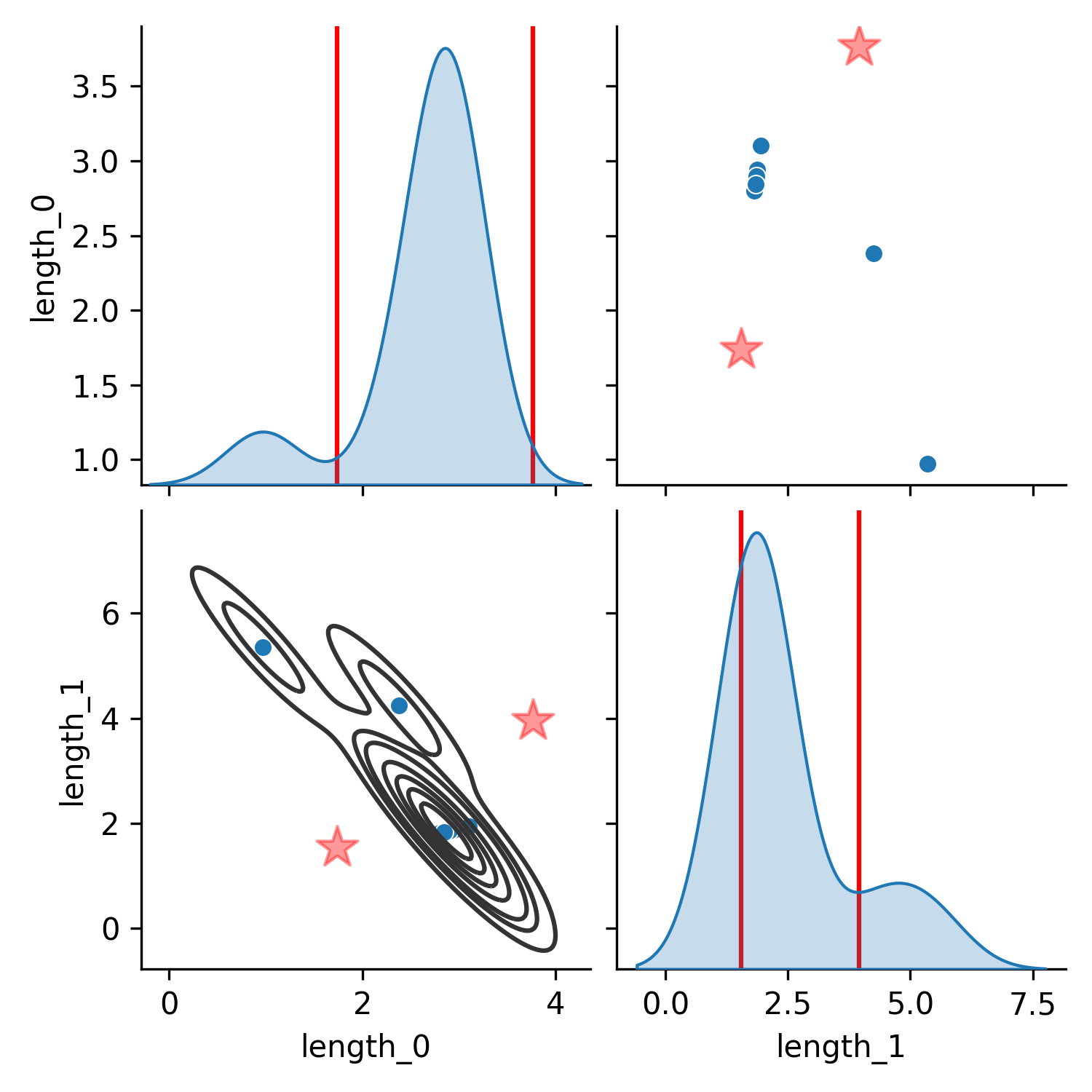(a) Product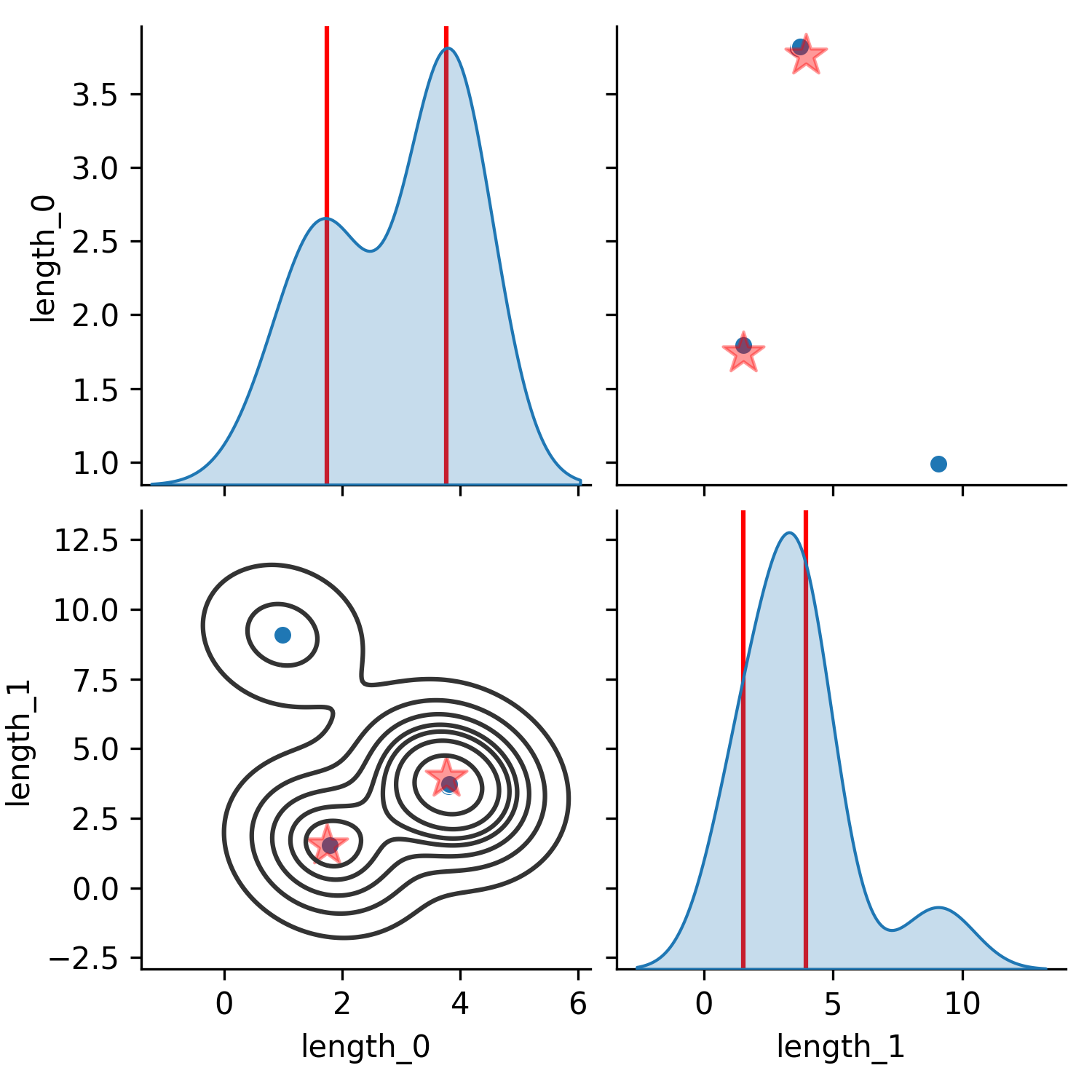(b) Sum

Figure 1. Comparison of posterior parameter distributions obtained from fitting the parameters to two ground-truth trajectories generated from different link lengths of a simulated double pendulum (units of the axes in meters). The trajectories were 300 steps long (which corresponds to a length of 3s) and contain the 2 joint positions and 2 joint velocities of the uncontrolled double pendulum which starts from a zero-velocity initial configuration where the first angle is at $90^\circ$ (sideways) and the other at $0^\circ$. In (a), the product of the individual per-trajectory likelihoods is maximized (Equation 2). In (b), the sum of the likelihoods is maximized (Equation 1).

#### 1.3 State and Parameter Normalization

The parameters we are estimating are valid over only particular ranges of values. These ranges are often widely different - in the case of our real-world pendulum experiment, the center of mass of a link in a pendulum may be in the order of centimeters, while the angular velocity at the beginning of the recorded motion can reach values on the orders of meters per second. It is therefore important to scale the parameters to a common range to avoid any dimension to dominate smaller parameter ranges during the estimation.

Similarly, the state dimensions are of different units - for example, we typically include velocities and positions in the recorded data over which we compute the likelihood. Therefore, we also normalize the range over the state dimensions. Given the state vector, respective parameter vector, $w$, we normalize each dimension $i$ by its statistical variance $\sigma^2$, i.e. $\frac{w_i}{\sigma_i^2}$.

#### 1.4 KNN-based Approximation for KL Divergence

In this work, we compare a set of parameter guesses (particles) to the ground-truth parameters, or a set of trajectories generated by simulating trajectories from each parameter in the particle distribution to a set of trajectories created on a physical system. To compare these distributions, we use the KL divergence to determine how the two distributions differ from each other. Formally, the KL divergence is the expected value of the log likelihood ratio between two distributions, and is an asymmetric divergence that does not satisfy the triangle inequality.

The KL divergence is easily computable in the case of discrete distributions or simple parametric distributions, but is not easily calculable for samples from non-parametric distributions such as those over trajectories. Instead, we use an approximation to the KL divergence which uses the relative distances between samples in a set to estimate the KL divergence between particle distributions. This method has been used to compare particle distributions over robot poses to asses the performance of particle filter distributions . To estimate the KL divergence between particle distributions over trajectories $\trajectoryset^{p}$ and $\trajectoryset^{q}$ we adopt the formulation from [4,3]: $$\begin{equation} \tilde{d}_{KL} (\trajectoryset^{p} \parallel \trajectoryset^{q}) = \frac{\observationdim}{|\trajectoryset^p|} \sum_{i=1}^{|\trajectoryset^p|} \log\frac{\operatorname{KNN}^p_{k_i}(i)}{\operatorname{KNN}^q_{l_i}(i)}+ \frac{1}{|\trajectoryset^p|} \sum_{i=1}^{|\trajectoryset^p|} [\psi(l_i) - \psi(k_i)] + \log\frac{|\trajectoryset^q|}{|\trajectoryset^p|-1}, \end{equation}$$ where $\observationdim$ is the dimensionality of the trajectories, $|\trajectoryset^p|$ is the number of trajectories in the $\trajectoryset^{p}$ dataset, $|\trajectoryset^q|$ is the number of particles in the $\trajectoryset^{q}$ dataset, $\operatorname{KNN}^p_{k_i}(i)$ is the distance from trajectory $\trajectory_i \in \trajectoryset^{p}$ to its $k_i$-th nearest neighbor in $\trajectoryset^{q}$, $\operatorname{KNN}^q_{l_i}(i)$ is the distance from trajectory $\trajectory_i \in \trajectoryset^{p}$ to its $l_i$-th nearest neighbor in $\trajectoryset^{p} \backslash \trajectory_i$, and $\psi$ is the digamma function. Note that this approximation of KL divergence can also be applied to compare parameter distributions, as we show in the synthetic data experiment from subsection 5.1 of our main paper (cf. Figure 4 (a) and (b) from the main paper) where the ground-truth parameter distribution is known.

Throughout this work, we set $k_i$ and $l_i$ to 3 as this reduces the bias in the approximation, but does not require a large amount of samples from the ground-truth distribution.

### 2 Experiments

We provide further technical details and results from the experiments we present in the main paper.

#### 2.1 Differentiable Simulator

Other than requiring a differentiable forward dynamics model which allows to simulate the system in its entirety following the Markov assumption, our proposed algorithm does not rely on a particular choice of dynamical system or simulator for which its parameters need to be estimated. For our experiments, we use the Tiny Differentiable Simulator  that implements end-to-end differentiable contact models and the Articulated Body Algorithm (ABA)  to compute the forward dynamics (FD) for articulated rigid-body mechanisms. Given joint positions $\jointpos$, velocities $\jointvel$, torques $\jointtorque$ in generalized coordinates, and external forces $\externalforce$, ABA calculates the joint accelerations $\jointacc$. We use semi-implicit Euler integration to advance the system dynamics in time for a time step $\Delta t$: \begin{align} \jointacc_{t+1} = \operatorname{ABA}(\jointpos_t, \jointvel_t, \jointtorque_t, \externalforce_t; \params), \qquad \jointvel_{t+1} = \jointvel_t + \jointacc_{t+1} \timestep, \qquad \jointpos_{t+1} = \jointpos_t + \jointvel_{t+1} \timestep. \end{align}

The second-order ODE described by Equation 4 is lowered to a first-order system, with state $\statevec_t = \begin{bmatrix}\jointpos_t & \jointvel_t\end{bmatrix}$. Furthermore, we deal primarily with the discrete time-stepped dynamics function $\statevec_{t+1} = \fstep(\statevec_t, t, \params)$, assuming that $\timestep$ is constant. The function $\fsim(\params, \statevec_0)$ uses $\fstep$ iteratively to produce a trajectory of states $[\statevec]_{t=1}^T$ given an initial state $\statevec_0$ and the parameters $\params$. Many systems of practical interest for robotics are controlled by an external input. In our formulation for parameter estimation, we include controls as explicit dependencies on the time parameter $t$.

For an articulated rigid body system, the parameters $\params$ may include (but are not limited to) the masses, inertial properties and geometrical properties of the bodies in the mechanism, as well as joint and contact friction coefficients. Given $\frac{\partial \fstep}{\partial\params}$ and $\frac{\partial\fstep}{\partial\statevec}$, gradients of simulation parameters with respect to the state trajectories can be computed directly through the chain rule, or via the adjoint sensitivity method .

#### 2.2 Identify Real-world Double Pendulum

We set up the double pendulum estimation experiment with the 11 parameters shown in Table 1 to be estimated. The state space consists of the two positions and velocities of both joints: $\statevec = \begin{bmatrix}\mathbf{q}_{0:1} & \mathbf{\dot{q}}_{0:1}\end{bmatrix}$. The dataset of trajectories contains image sequences (see time lapse of an excerpt from a trajectory in Figure 2) and annotated pixel coordinates of the three vertices in the double pendulum, from which we extracted joint positions and velocities (via finite differencing given the known recording frequency of 400Hz).

Link 1 Mass 0.05 $\kg$ 0.5 $\kg$
$I_{xx}$ 0.002 $\kg\cdot\meter^2$ 1.0 $\kg\cdot\meter^2$
COM $x$ -0.2 $\meter$ 0.2 $\meter$
COM $y$ -0.2 $\meter$ 0.2 $\meter$
Joint friction 0.0 0.5
Link 2 Length 0.08 $\meter$ 0.3 $\meter$
Mass 0.05 $\kg$ 0.5 $\kg$
$I_{xx}$ 0.002 $\kg\cdot\meter^2$ 1.0 $\kg\cdot\meter^2$
COM $x$ -0.2 $\meter$ 0.2 $\meter$
COM $y$ -0.2 $\meter$ 0.2 $\meter$
Joint friction 0.0 0.5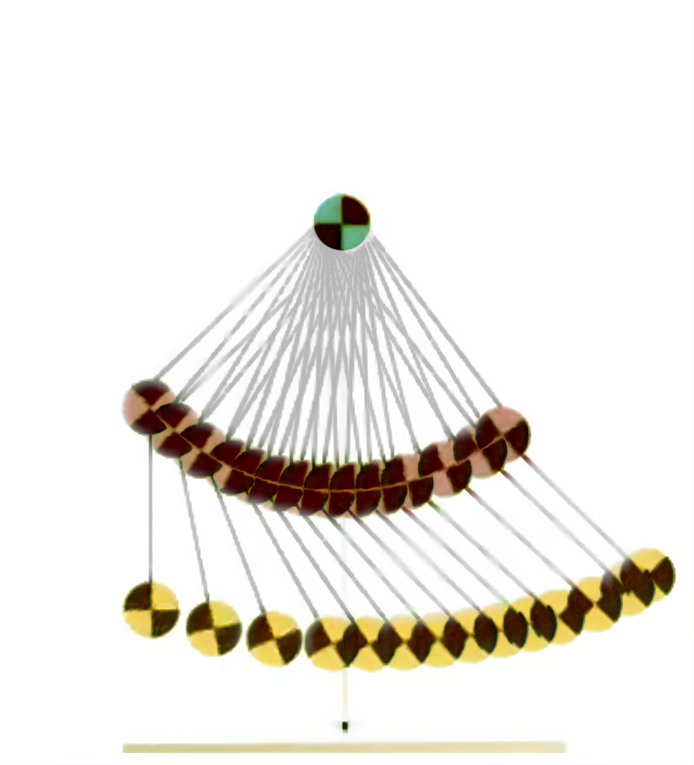Table 1. Parameters to be estimated. $I$ refers to the $3\times3$ inertia matrix, COM stands for center of mass.

Figure 2. Time lapse of a double pendulum trajectory from the IBM dataset .

Since we know that all trajectories in this dataset stem from the same double pendulum , we only used a single reference trajectory as target trajectory $\trajectory\real$ during the estimation. We let each estimator run for 2000 iterations. For evaluation, we calculate the consistency metrics from Tab. 1 of the main paper over 10 held-out trajectories from a test dataset. For comparison, we visualize the trajectory density over simulations rolled out from the last 100 Markov samples (or 100 particles in the case of particle-based approaches) in Figure 3. The ground-truth shown in these plots again stems from the unseen test dataset. This experiment further demonstrates the generalizability of simulation-based inference, which, when an adequate model has been implemented and its parameters identified, can predict outcomes under various novel conditions even though the training dataset consisted of only a single trajectory in this example.

 Emcee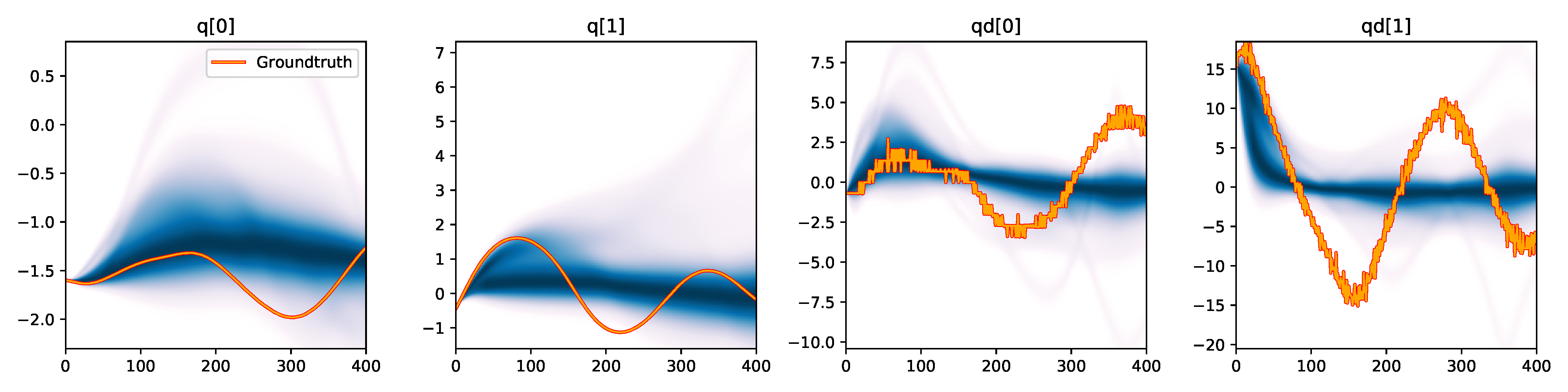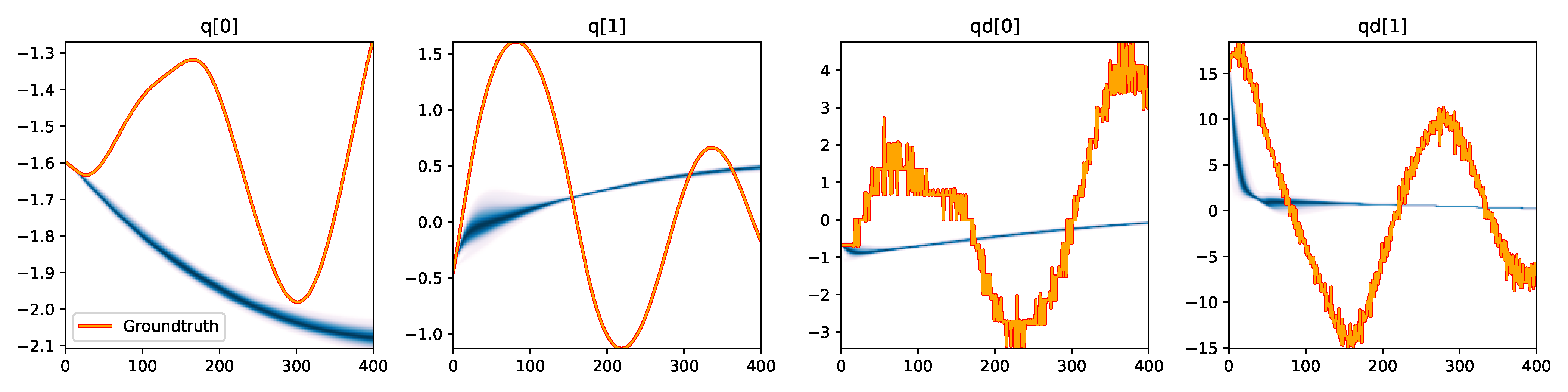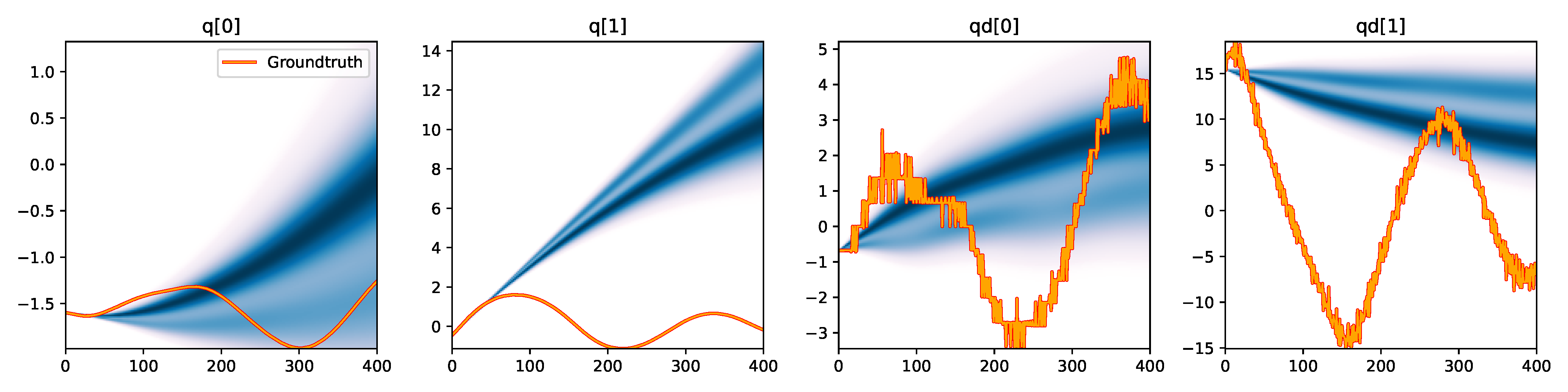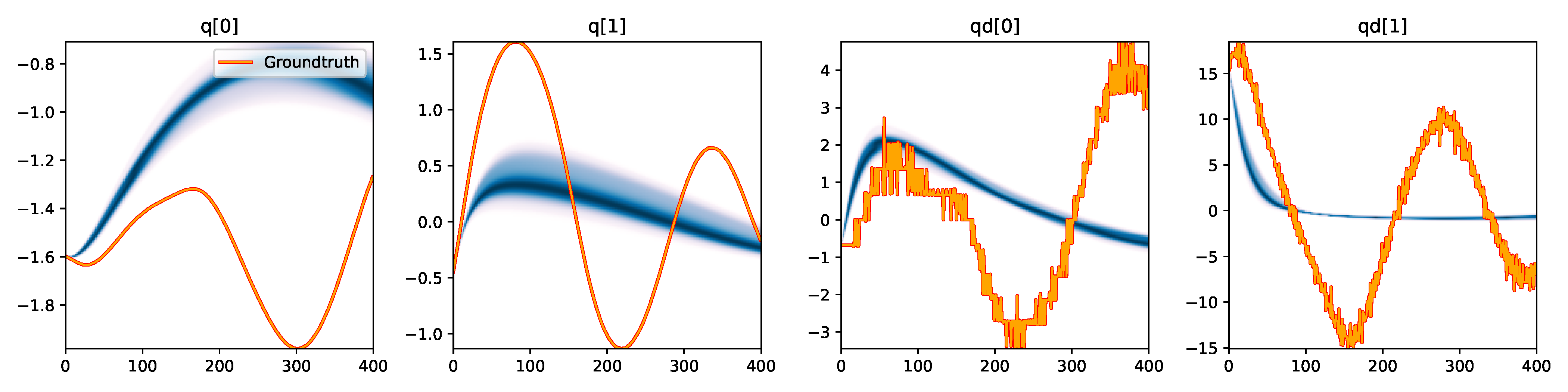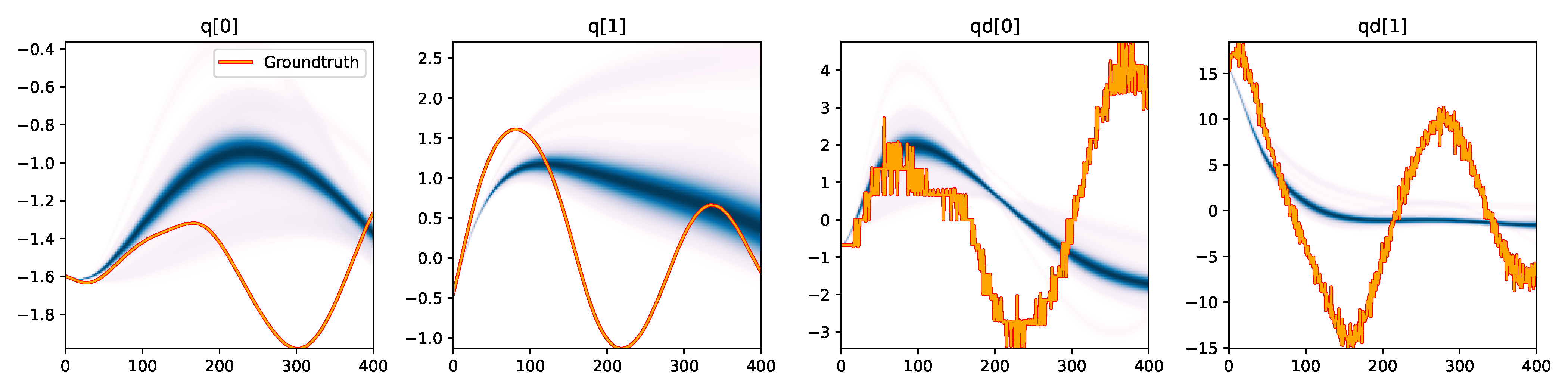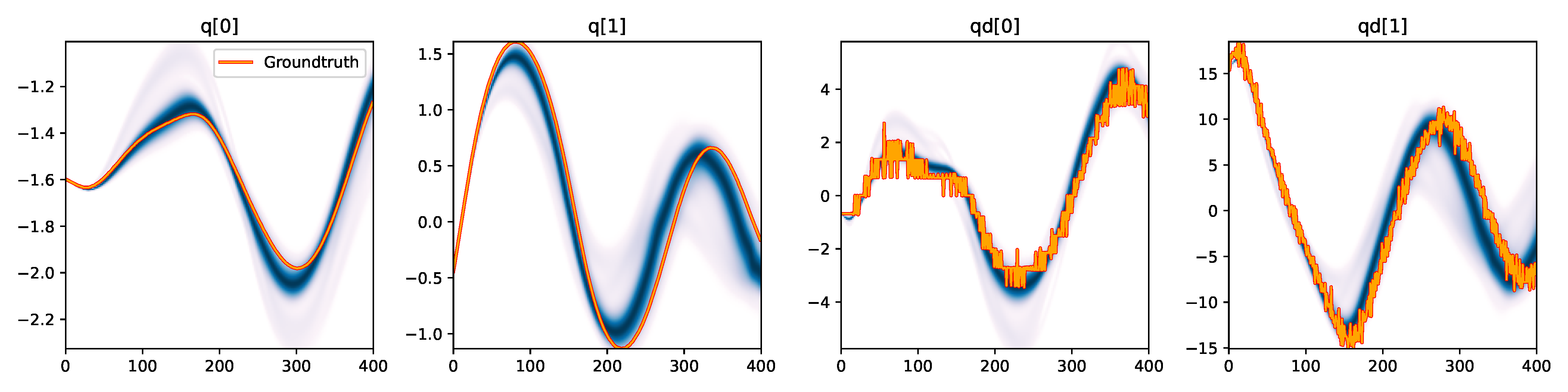Figure 3. Kernel density estimation over trajectory roll-outs from the last estimated 100 parameter guesses of each method, applied to the physical double pendulum dataset from Section 2.2. The ground-truth trajectory here stems from the test dataset of 10 trajectories that were held out during training. The particle-based approaches (CEM, SVGD, CSVGD) use 100 particles.

#### 2.3 Ablation Study on Multiple Shooting

We evaluate the baseline estimation algorithms with our proposed multiple-shooting likelihood function (using 10 shooting windows) on the physical double pendulum dataset from before. To make the constrained optimization problem amenable to the MCMC samplers, we formulate the defect constraint through the following likelihood: \begin{align} \pdef(\statevec_t^s, \statevec_t) = \mathcal{N}(\statevec_t^s | \statevec_t,\sigma^2_{\text{def}}) \qquad t\in [h, 2h, \dots], \end{align} where we tune $\sigma^2_{\text{def}}$ to a small value (on the order of $10^{-2}$) such that the defects are minimized during the estimation. As we describe in Sec. 4.4 from our main paper, the parameter space is augmented by the shooting variables $\statevec_t^s$.

As shown in Table 2, the MCMC approaches Emcee and NUTS do not benefit meaningfully from the multiple-shooting approach. Emcee often yields unstable simulations from which we are not able to compute some of the metrics. The increased dimensionality of the parameter space appears to add a significant challenge to these methods, which are known to scale poorly to higher dimensions. Despite being configured to use a Gaussian mixture model of 3 kernels, the CEM posterior immediately collapses to a single point such that the KL divergence of simulated against real trajectories cannot be computed.

$d_{\text{KL}} (\trajectoryset\real \parallel \trajectoryset\simu)$ $d_{\text{KL}} (\trajectoryset\simu \parallel \trajectoryset\real)$ MMD
Algorithm SS MS SS MS SS MS
Emcee 8542.2466 8950.4574 4060.6312 N/A 1.1365 N/A
CEM 8911.1798 8860.5115 8549.5927 N/A 0.9687 0.5682
SGLD 8788.0962 5863.2728 7876.0310 2187.2825 2.1220 0.0759
NUTS 9196.7461 8785.5326 6432.2131 4935.8983 0.5371 1.1642
(C)SVGD 8803.5683 5204.5336 10283.6659 2773.1751 0.7177 0.0366

Table 2. Consistency metrics of the posterior distributions approximated from the physical double pendulum dataset (Section 2.2) by the different estimation algorithms using the single-shooting likelihood $p_{ss}(\trajectory\real | \params)$ (column "SS") and the multiple-shooting likelihood $p_{ms}(\trajectory\real | \params)$ (column "MS") with 10 shooting windows. Note that SVGD with multiple-shooting corresponds to CSVGD.

We observe a significant improvement in estimation accuracy on SGLD, where the multiple-shooting approach allowed it to converge to closely matching trajectories, as shown in Figure 4. As with SVGD, the availability of gradients allows this method to scale better to the higher dimensional parameter space, while the smoothed likelihood landscape further helps the approach to find better fitting parameters.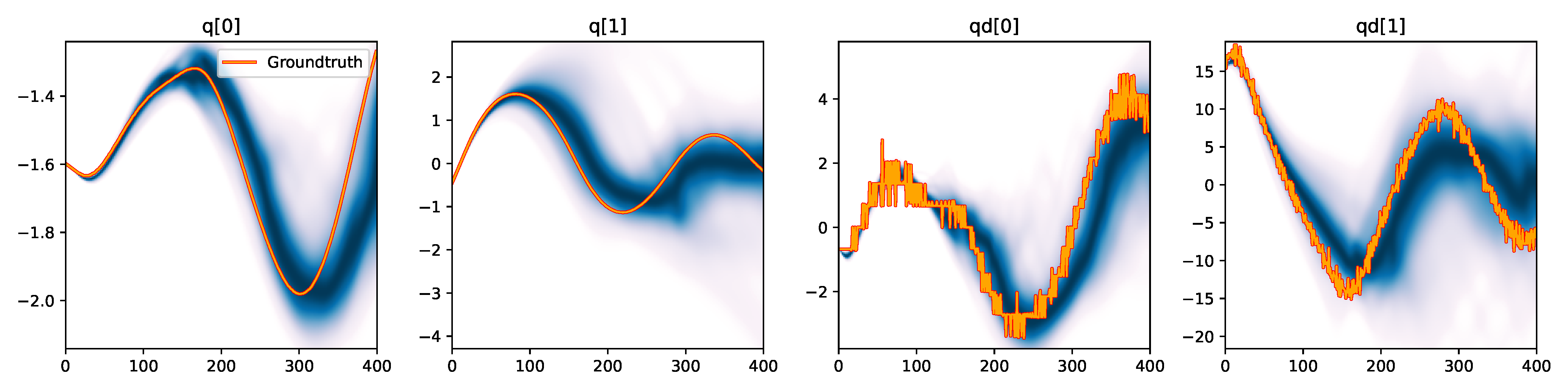Figure 4. Kernel density estimation over trajectory roll-outs from the last estimated 100 parameter guesses of SGLD with the multiple-shooting likelihood model (see Section 2.3), applied to the physical double pendulum dataset from Section 2.2. Similarly to SVGD, SGLD benefits significantly from the smoother likelihood function while being able to cope with the augmented parameter space thanks to its gradient-based approach.

#### 2.4 Comparison to Likelihood-free Inference

Our Bayesian inference approach leverages the simulator as part of the likelihood model to approximate posterior distributions over simulation parameters, which means the simulator is indispensable in our estimation process}. In the following, we compare our approach against the likelihood-free inference approach BayesSim  that leverages approximate Bayesian computation (ABC) which is the most popular family of algorithms within likelihood-free methods.

Likelihood-free methods assume the simulator is a black box that can generate a trajectory given a parameter setting. Instead of querying the simulator to evaluate the likelihood (as in our approach), a conditional density $q(\params | \trajectoryset)$ is learned directly from a dataset of simulation parameters and their corresponding rolled-out trajectories via supervised learning to approximate the posterior. A common choice of model for such density is a mixture density network , which parameterizes a Gaussian mixture model. This is in contrast to our (C)SVGD algorithm which can approximate any shape of posterior by being a nonparametric inference algorithm.

For our experiments we collect a dataset of 10,000 simulated trajectories of parameters randomly sampled from the prior distribution. We train the density network via the Adam optimizer with a learning rate of $10^{-3}$ for 3000 epochs, after which we observed no meaningful improvement to the calculated log-likelihood loss during training. In the following, we provide further details on the likelihood-free inference pipeline we consider, by describing the input data processing and the model used for approximating the posterior.

##### 2.4.1 Input Data Processing

The input to the learned density model has to be a sufficient statistic of the underlying data, while being low-dimensional in order to keep the learning problem computationally tractable. We consider the following four methods of processing the trajectories that are the input to the likelihood-free methods, as visualized for an example trajectory in Figure 5. Note that we configured the following input processing methods to generate a one-dimensional input vector that has a reasonable length to be computationally feasible to train on (given the 10,000 trajectories from the training dataset), while achieving acceptable performance which we validated through testing various settings.

Downsampled: we down-sample the trajectory so that for the double pendulum experiment (Section 2.2) we use only every 20th state, for the Panda arm experiment (Section 2.5) only every 200-th state of the trajectory. Finally, the state dimensions per trajectory are concatenated to a one-dimensional vector.

Difference: we adapt the input statistic from the original BayesSim approach in [9, Eq. (22)] where the differences of two consecutive states along the trajectory are used in concatenation with their mean and variance: $$\psi(\trajectory) = (\operatorname{downsample}(\tau), \mathbb{E}[\tau], \operatorname{Var}[\tau]) \qquad\text{where}\qquad \tau = \{\statevec_t - \statevec_{t-1}\}_{t=1}^T$$ As before, we down-sample these state differences and concatenate them to a vector.

Summary: for each state dimension of the trajectory, we compute the following statistics typical for time series: mean, variance, cross correlation between state dimensions of the trajectory, as well as auto-correlations for each dimension at 5 different time delays: [5, 10, 20, 50, 100] time steps. These numbers are concatenated for all state dimensions to a one-dimensional vector per input trajectory.

Signature: we compute the signature transform from the signatory package  over the input trajectory. Such so-called path signatures have been recently introduced to extract information about order and area, thereby preserving features inherent to nonlinear trajectories. We select a depth for the signature transform of 3 for the double pendulum experiment, and 2 for the Panda arm experiment, to obtain feature vectors of comparable size to the aforementioned input techniques.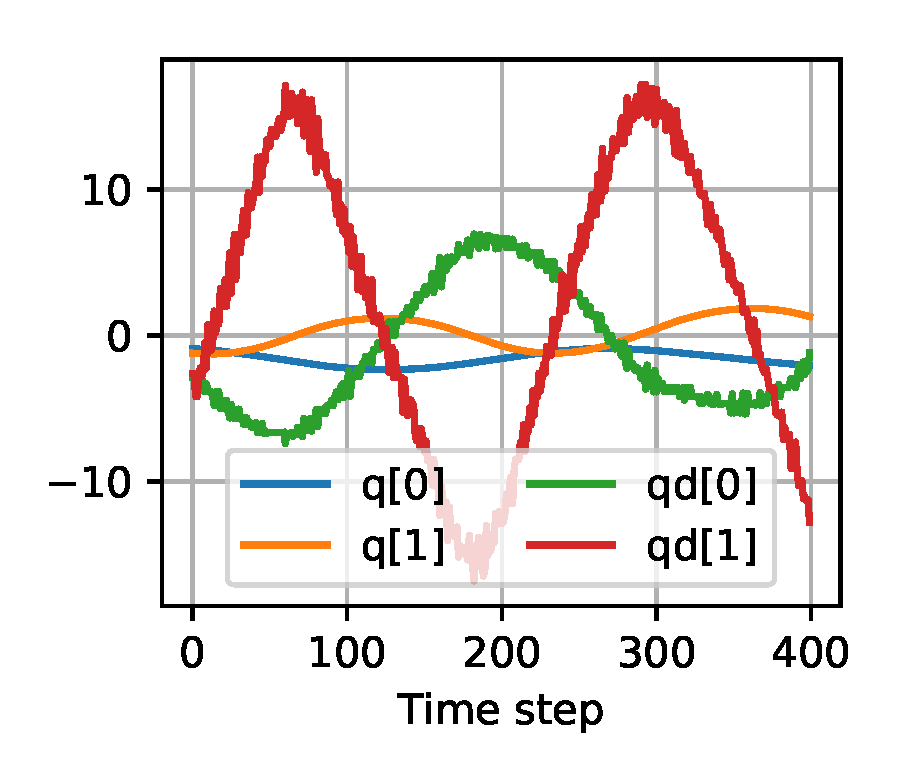(a) Raw Input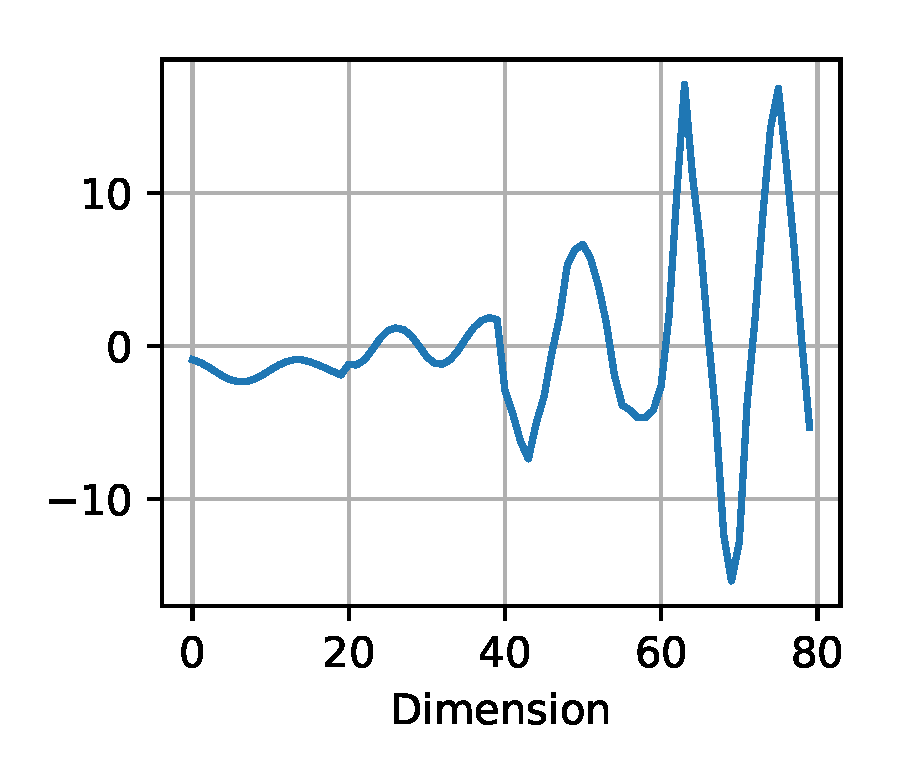(b) Downsampled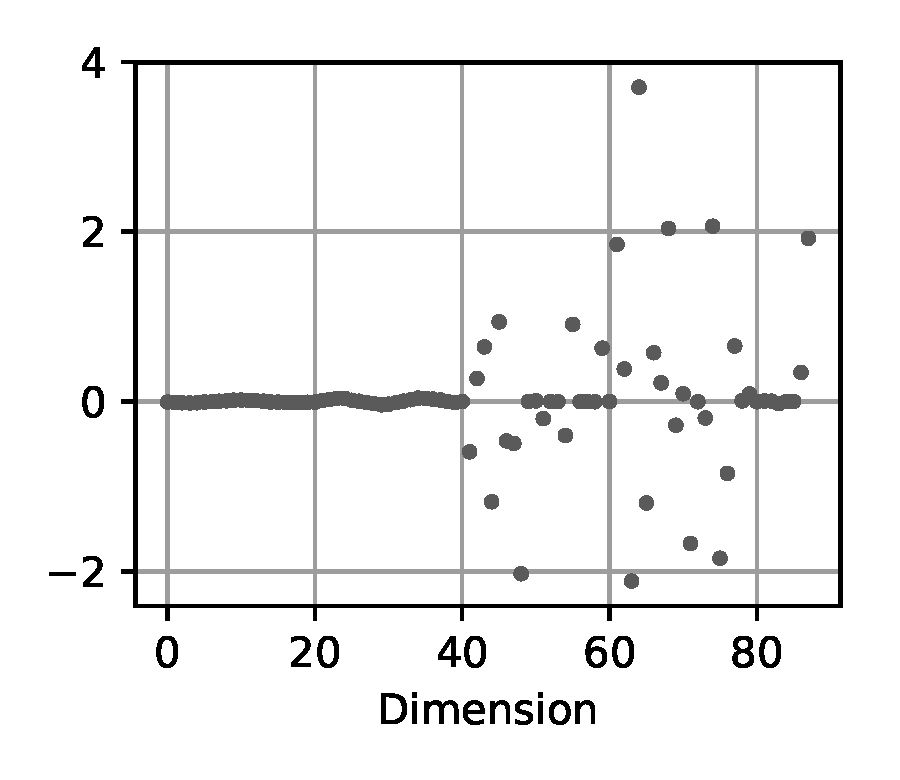(c) Difference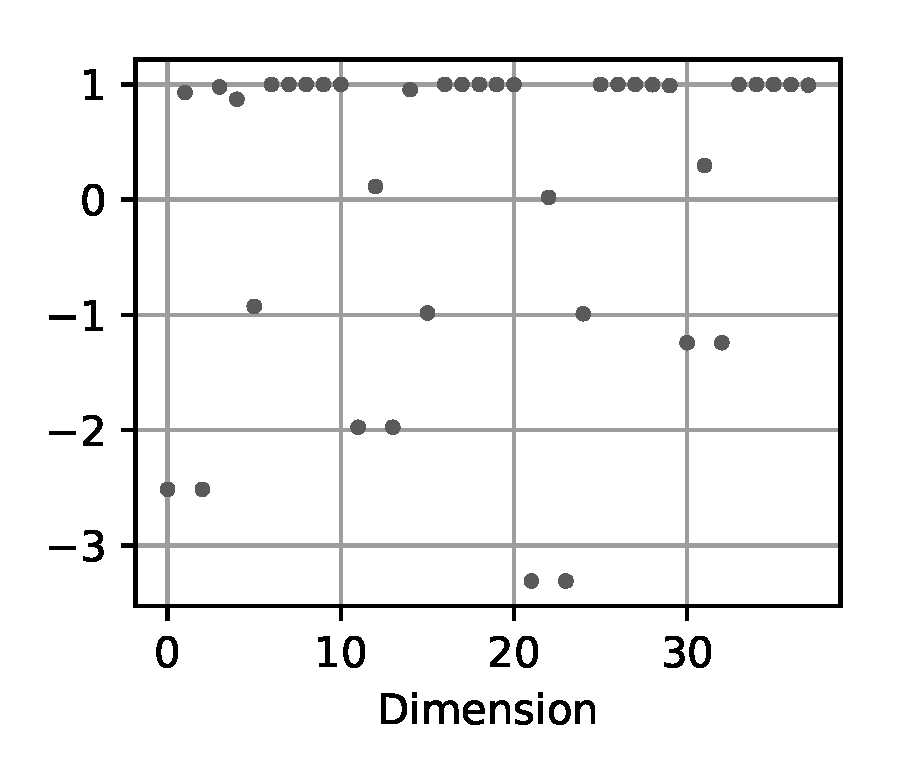(d) Summary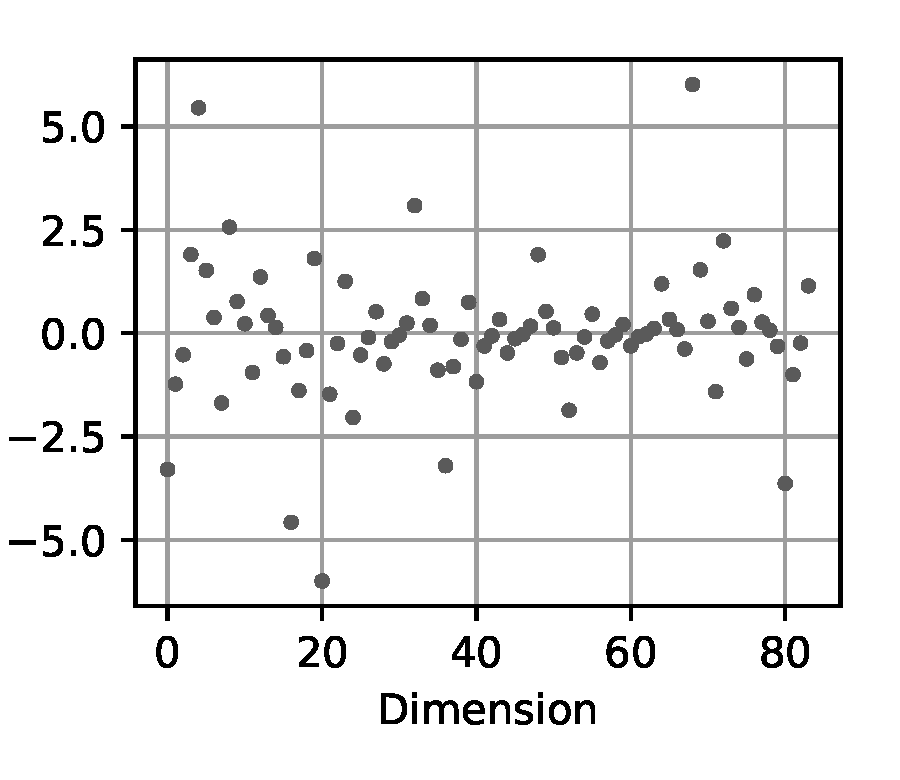(e) Signature

Figure 5. Exemplary visualization of the input processing methods for the likelihood-free baselines from Section 2.4 applied to a trajectory from the double pendulum experiment in Section 2.2.

##### 2.4.2 Density Model

As the density model for the learned posterior $q(\params | \trajectoryset)$, we select the following commonly used representations.

Mixture density network (MDN): uses neural network features from a feed-forward neural network using two hidden layers with 24 units each.

Mixture density random Fourier features (MDRFF): this density model uses Fourier features and a kernel. We evaluate the MDRFF with the following common choices for the kernel:

• Radial Basis Function (RBF) kernel
• Matérn kernel [12, Eq. (4.14)] with $\nu=\frac{5}{2}$
##### 2.4.3 Evaluation

Note that instead of action generation, which is part of the proposed BayesSim pipeline , we only focus on the inference of the posterior density over simulation parameters in order to compare such likelihood-free inference approach against our method.

To evaluate the metrics shown in Table 2 for each BayesSim instantiation (input method and density model), we sample 100 parameter vectors from the learned posterior $q(\params | \trajectoryset)$ and simulate them to obtain 100 trajectories which are compared against the reference trajectory sets, as we did in the comparison for the other Bayesian inference methods in Tab. 1 of the main paper.

Double Pendulum Experiment Panda Arm Experiment Input Model 8817.9222 4050.4666 0.6748 -17.4039 8919.2463 4633.2637 0.6285 -17.1646 9092.5575 5093.8851 0.5664 -18.3481 8985.8056 4610.5438 0.5807 -19.3432 9027.9474 5091.5283 0.5593 -17.2335 8936.3823 4282.8599 0.5988 -18.4892 9063.1753 4884.1398 0.5672 -19.5430 8980.9080 4081.1160 0.6016 -18.3458 8818.1830 3794.9873 0.6110 -17.6395 8859.2156 4349.9971 0.6176 -17.2752 8962.0501 4241.4551 0.5999 -19.6672 9036.9626 4620.9517 0.5715 -18.1652 5204.5336 2773.1751 0.0366 -15.1671

Table 2. Consistency metrics of the posterior distributions approximated by the different BayesSim instantiations, where the input method and model name (including the kernel type for the MDRFF model) are given. Each metric is calculated across simulated and real trajectories. Lower is better on all metrics except the log-likelihood $\log\pobs(\trajectoryset\real \parallel \trajectoryset\simu)$ from the Panda arm experiment. For comparison, in the last row, we reproduce the numbers from CSVGD shown in Tab. 1 of the main paper.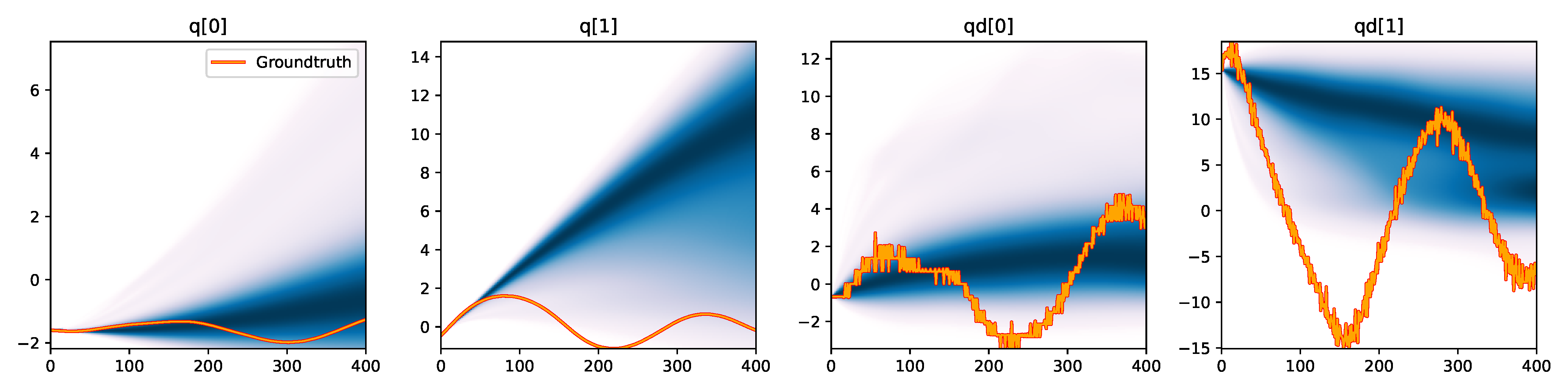MDN - Downsampled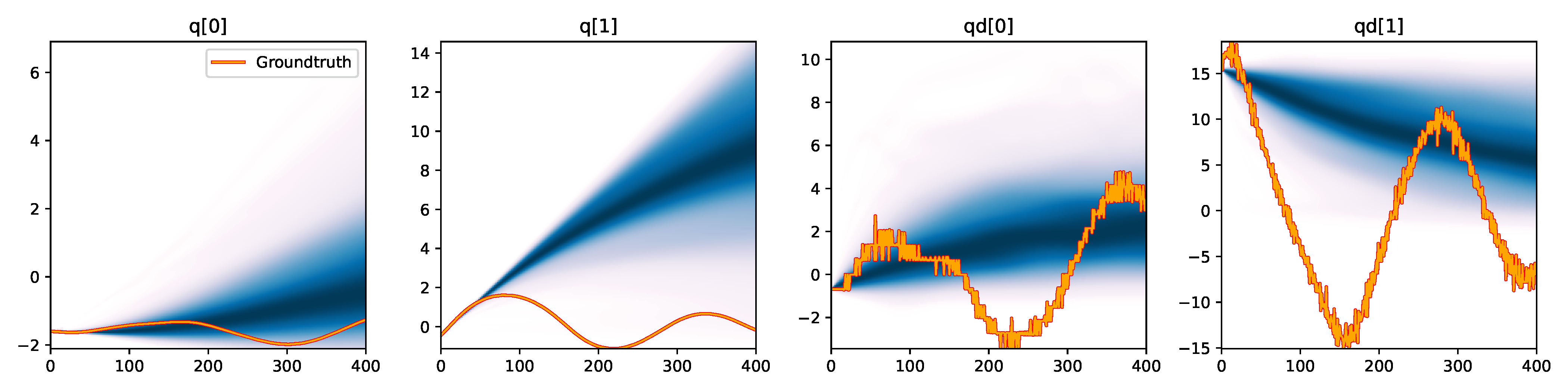MDN - Difference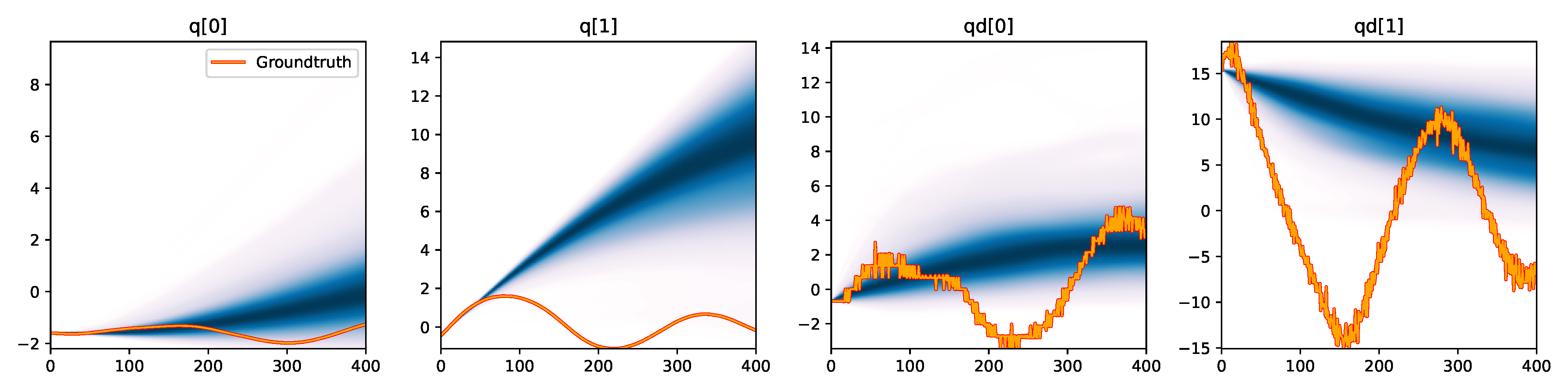MDN - Summary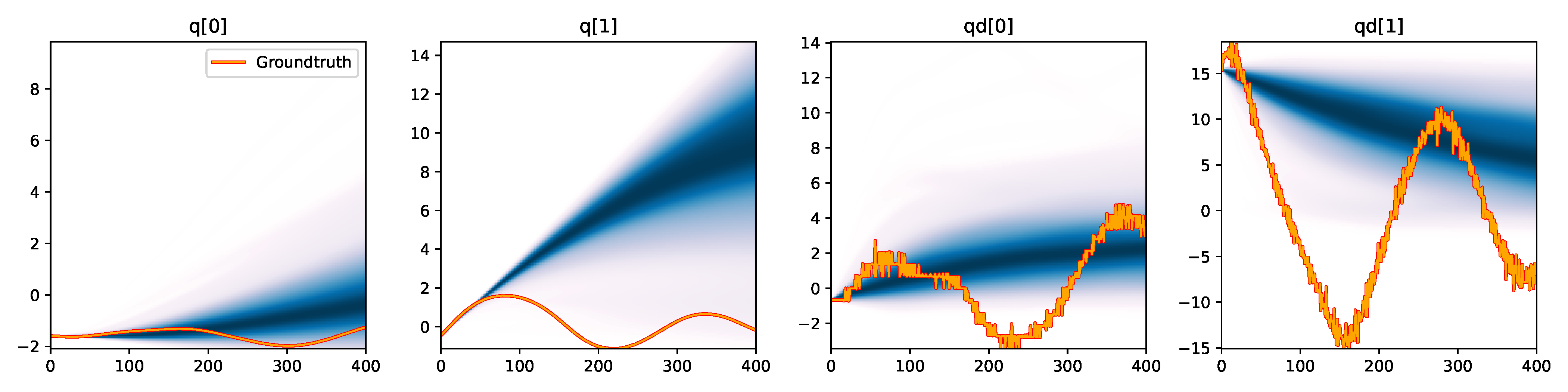MDN - Signature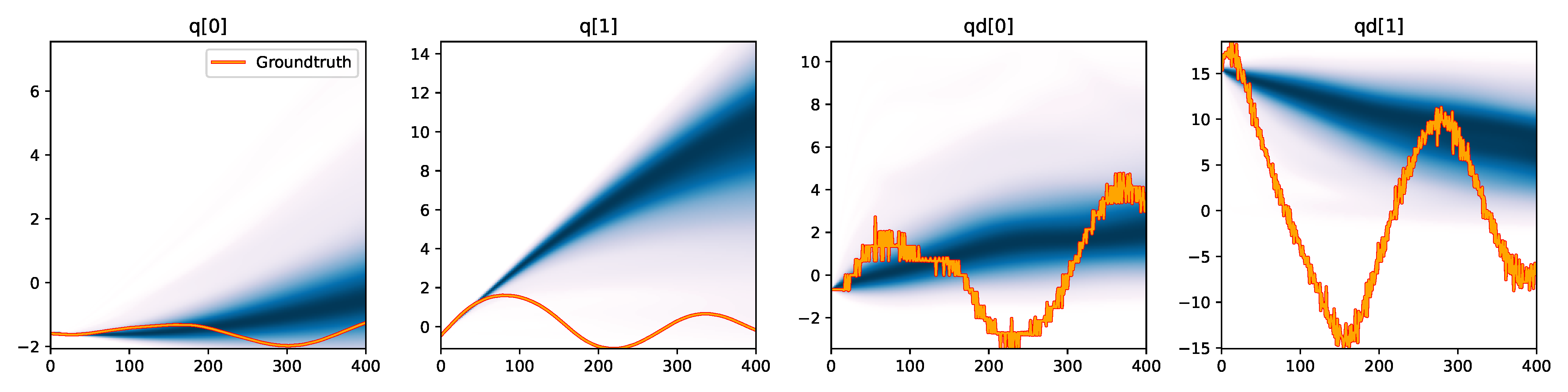MDRFF (RBF) - Downsampled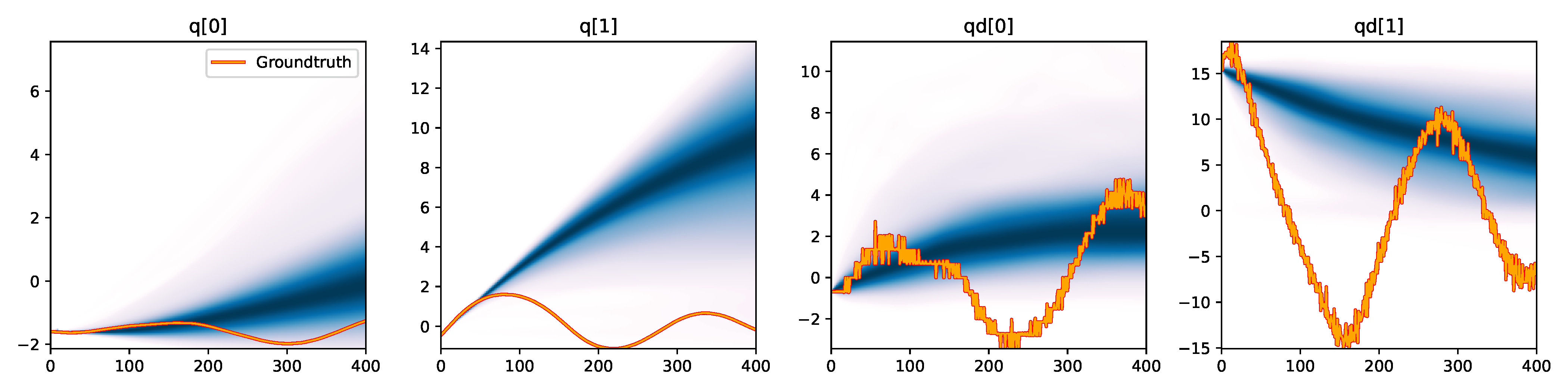MDRFF (RBF) - Difference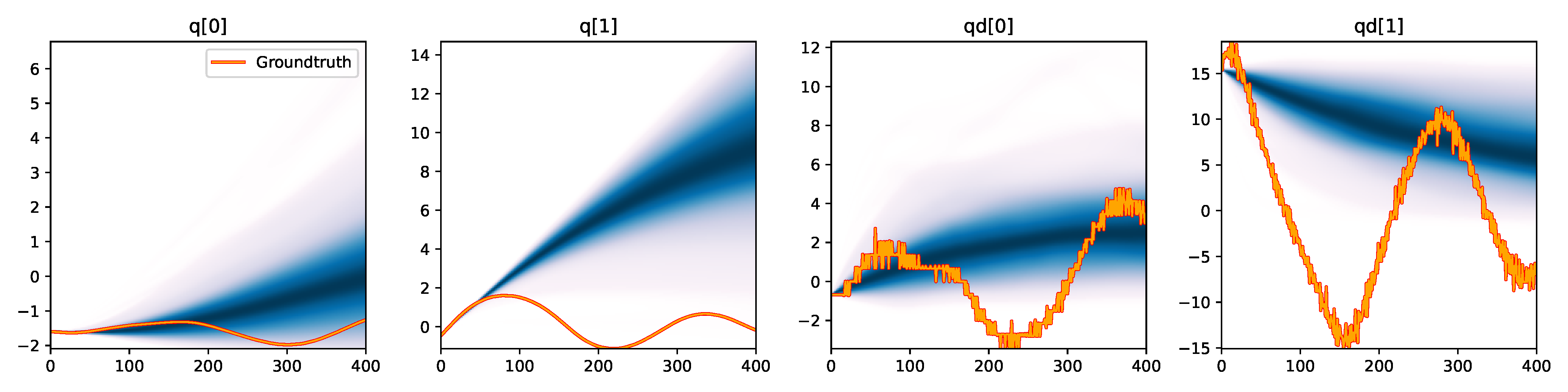MDRFF (RBF) - Summary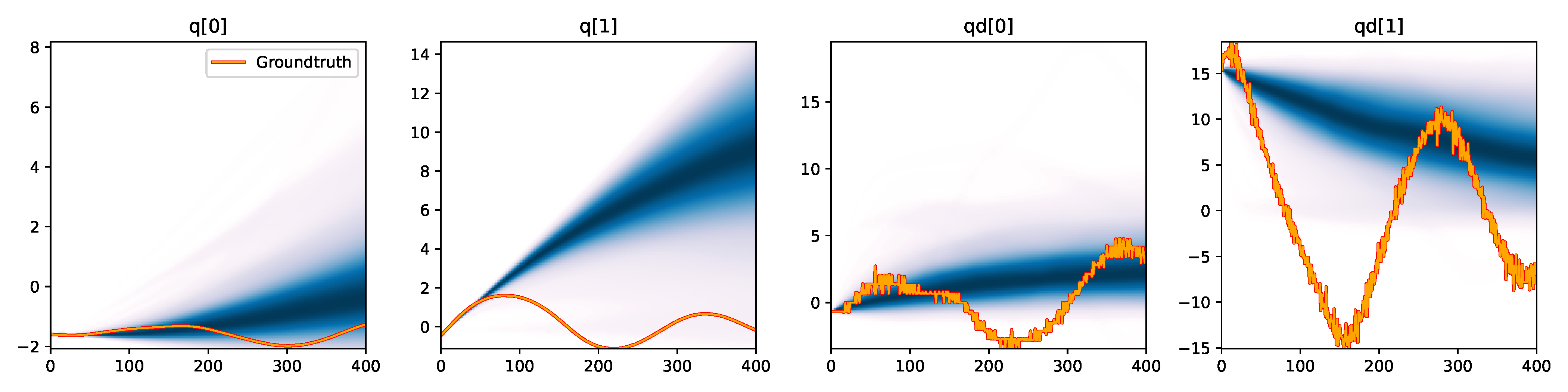MDRFF (RBF) - Signature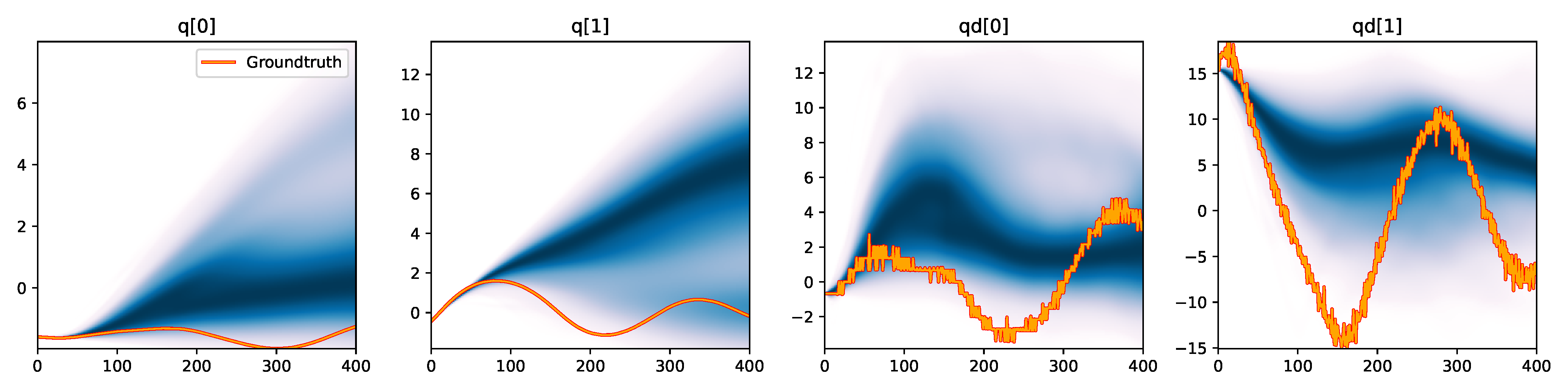MDRFF (Matérn) - Downsampled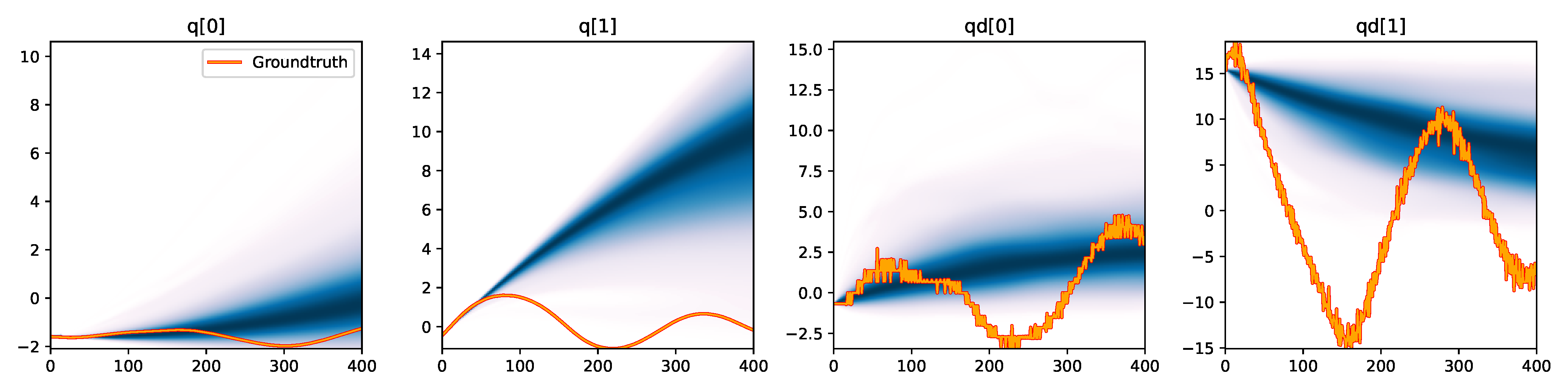MDRFF (Matérn) - Difference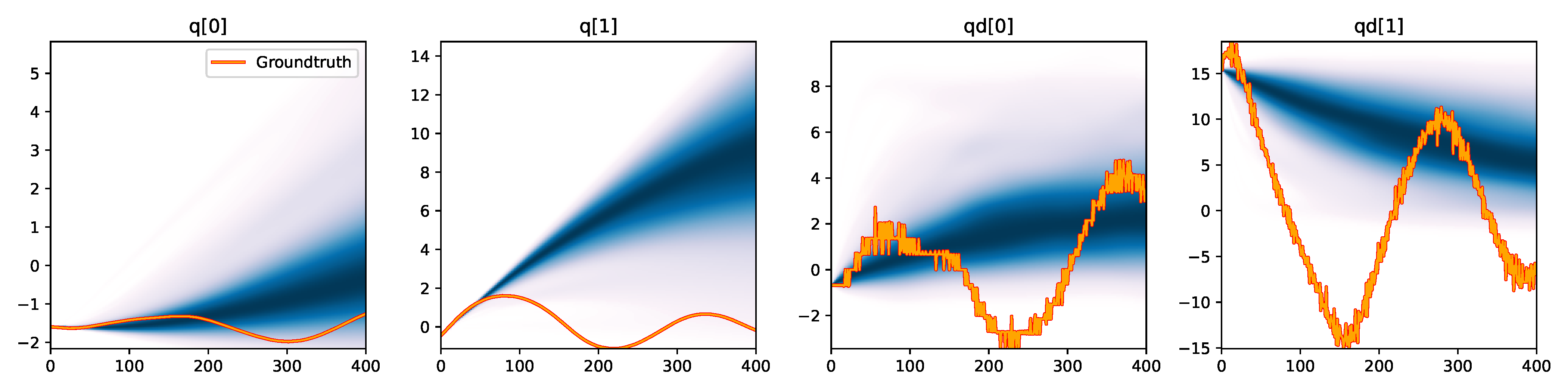MDRFF (Matérn) - Summary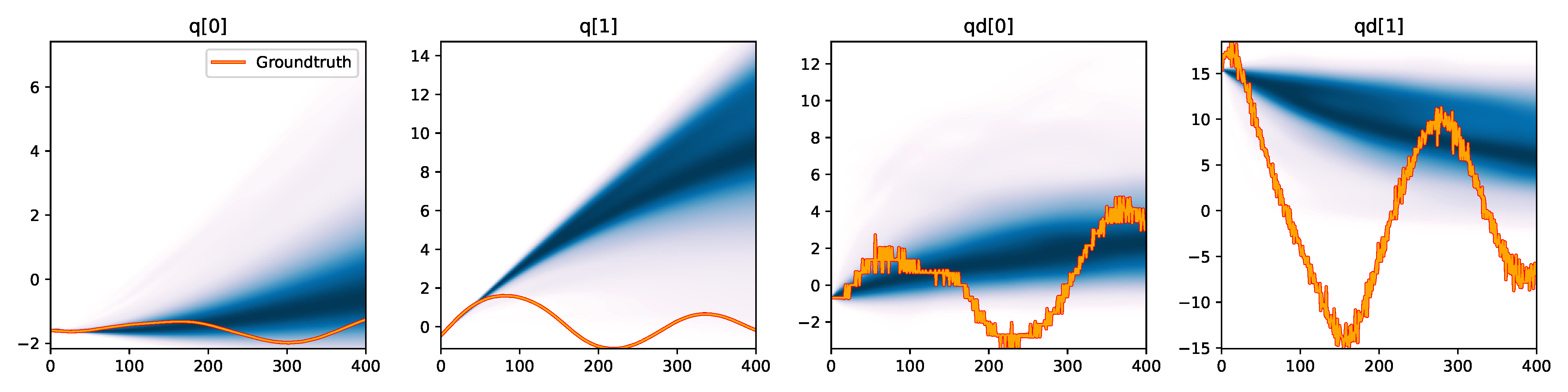MDRFF (Matérn) - Signature

Figure 6. Kernel density estimation over trajectory roll-outs from 100 parameter samples drawn from the posterior of each BayesSim method (model name with kernel choice in bold font + input method, see Section 2.4), applied to the physical double pendulum dataset from Section 2.2. The ground-truth trajectory here stems from the test dataset of 10 trajectories that were held out during training.

##### 2.4.4 Discussion

The results from our experiments with the various likelihood-free approaches in Table 2 indicate that, among the tested pipelines, the MDRFF model with Matérn kernel and downsampled trajectory input overall performed the strongest, followed by the MDN with downsampled input. In comparison to the likelihood-based algorithms from Tab. 1 of the main paper, these results are comparable on the double pendulum experiment. However, in comparison to CSVGD, the estimated likelihood-free posteriors are significantly less accurate, which can also be clearly seen in the density plots over the rolled out trajectories from such learned densities in Figure 6. On the Panda arm experiment, the likelihood-free methods are outperformed by the likelihood-based algorithms (such as the Emcee sampler) more often on the likelihood of the learned parameter densities. CSVGD again achieves a much more accurate posterior in this experiment than any likelihood-free approach.

Why do these likelihood-free methods perform so poorly on a seemingly simple double pendulum?

One would expect that this kind of dynamical system poses no greater challenge to BayesSim when it was shown to identify a cartpole's link length and cart mass successfully . To investigate this problem, we revisit the simplified double pendulum estimation experiment from Sec. 5.1 of our main paper, where only the two link lengths need to be estimated from simulated trajectories. As before, we create a dataset with 10,000 trajectories of 400 time steps based on the two simulation parameters sampled from a uniform distribution ranging between 0.5m and 5m. While keeping all parameters the same as in our previous double-pendulum experiment where eleven parameters had to be inferred, all of the density models in combination with both the "difference" and "downsampled" input statistic infer a highly accurate parameter distribution, as shown in Figure 7 (a). The trajectories produced by sampling from the BayesSim posterior (Figure 7 (b)) also match the reference observations much more closely than any of the BayesSim models on the previous 11-parameter double pendulum (Figure 6). These results suggest that BayesSim and potentially other likelihood-free method have problems in inferring higher dimensional parameter distributions. The experiments in  demonstrated as many as four parameters being estimated (for the acrobot), while showing inference results for simulated systems only. While our double pendulum system from Section 2.2 is basic in principle, the higher dimensional parameter space (see parameters in Table 1) and the fact that we need to fit against real-world data makes it a significantly harder problem for most state-of-the-art inference algorithms. CSVGD is able to achieve a close fit thanks to the multiple-shooting segmentation of the trajectory which improves the convergence (see more ablation results for multiple-shooting on this experiment in Section 2.3).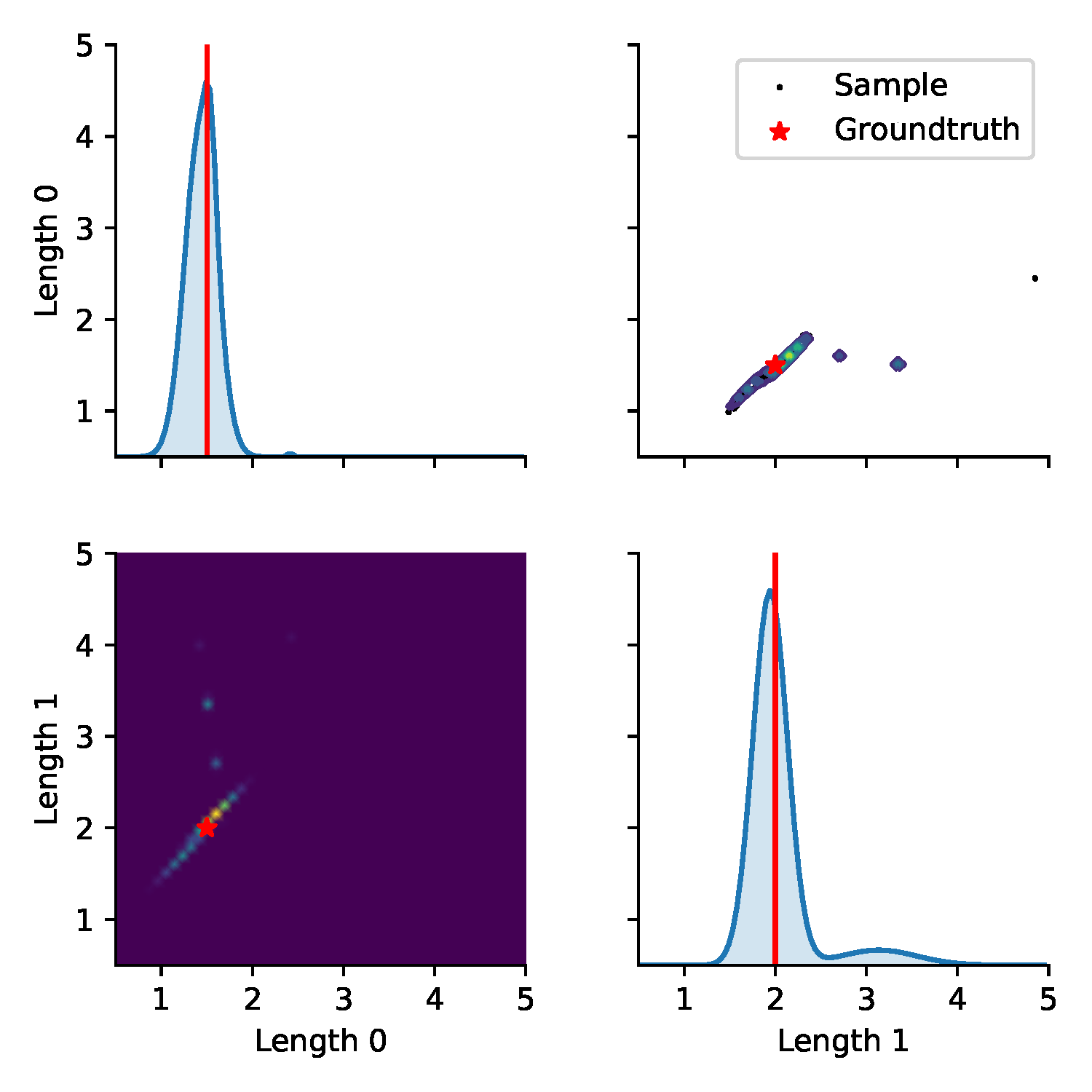(a) Posterior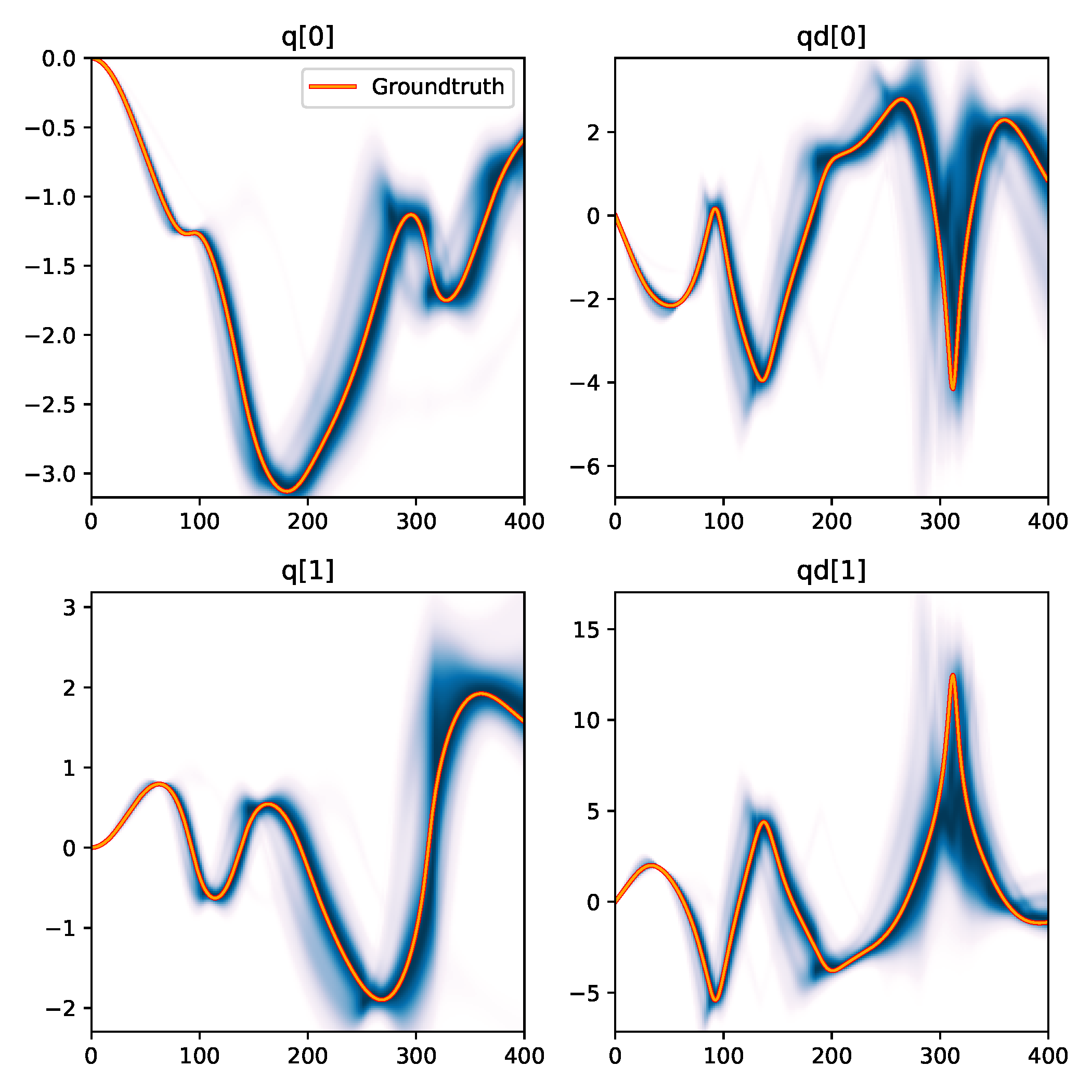(b) Trajectory density

Figure 7. Results from BayesSim on the simplified double pendulum experiment where only the two link lengths need to be inferred. (a) shows the approximated posterior distribution by the MDN model and "downsampled" input statistics. The diagonal plots show the marginal parameter distributions, the bottom-left heatmap and the top-right contour plot show the 2D posterior where the ground-truth parameters at (1.5m, 2m) are indicated by a red star. The black dots in the top-right plot are 100 parameters sampled from the posterior which are rolled out to generate trajectories for the trajectory density plot in (b). (b) shows a kernel density estimation over these 100 trajectories for the four state dimensions $(\jointpos_{[0:1]},\jointvel_{[0:1]})$ of the double pendulum.

#### 2.5 Identify Inertia of an Articulated Rigid Object

The state space consists of the positions and velocities of the seven degrees of freedom of the robot arm and the two degrees of freedom in the universal joint, resulting in a 20-dimensional state vector $\statevec=\begin{bmatrix}\mathbf{q}_{0:8} & \mathbf{\dot{q}}_{0:8} & \mathbf{q}^d_{0:6} & \mathbf{\dot{q}}^d_{0:6}\end{bmatrix}$ consisting of nine joint positions and velocities, plus the PD control position and velocity targets, $\mathbf{q}^d$ and $\mathbf{\dot{q}}^d$, for the actuated joints of the robot arm. We control the arm using the MoveIt! motion planning framework  by moving joints 6 and 7 to predefined joint-space offsets of $0.1$ and $-0.1$ radians, in sequence. We use the default Iterative Parabolic Time Parameterization algorithm with a velocity scaling factor of $0.1$. We track the motion of the acrylic box via a Vicon motion capture system and derive four Cartesian coordinates as observation $\observationvec=\begin{bmatrix}\mathbf{p}_{o} & \mathbf{p}_{x} & \mathbf{p}_{y} & \mathbf{p}_{z}\end{bmatrix}$ to represent the frame of the box (shown in Figure 8): a point of origin located at the center of the upper lid of the box, and three points located 1m away from the origin into the x, y, and z direction (transformed by the reference frame of the box). We chose this state representation to ease the computation of the likelihood, since we only need to compute differences between 3D points instead of computing the distances over 3D rotations which requires special treatment .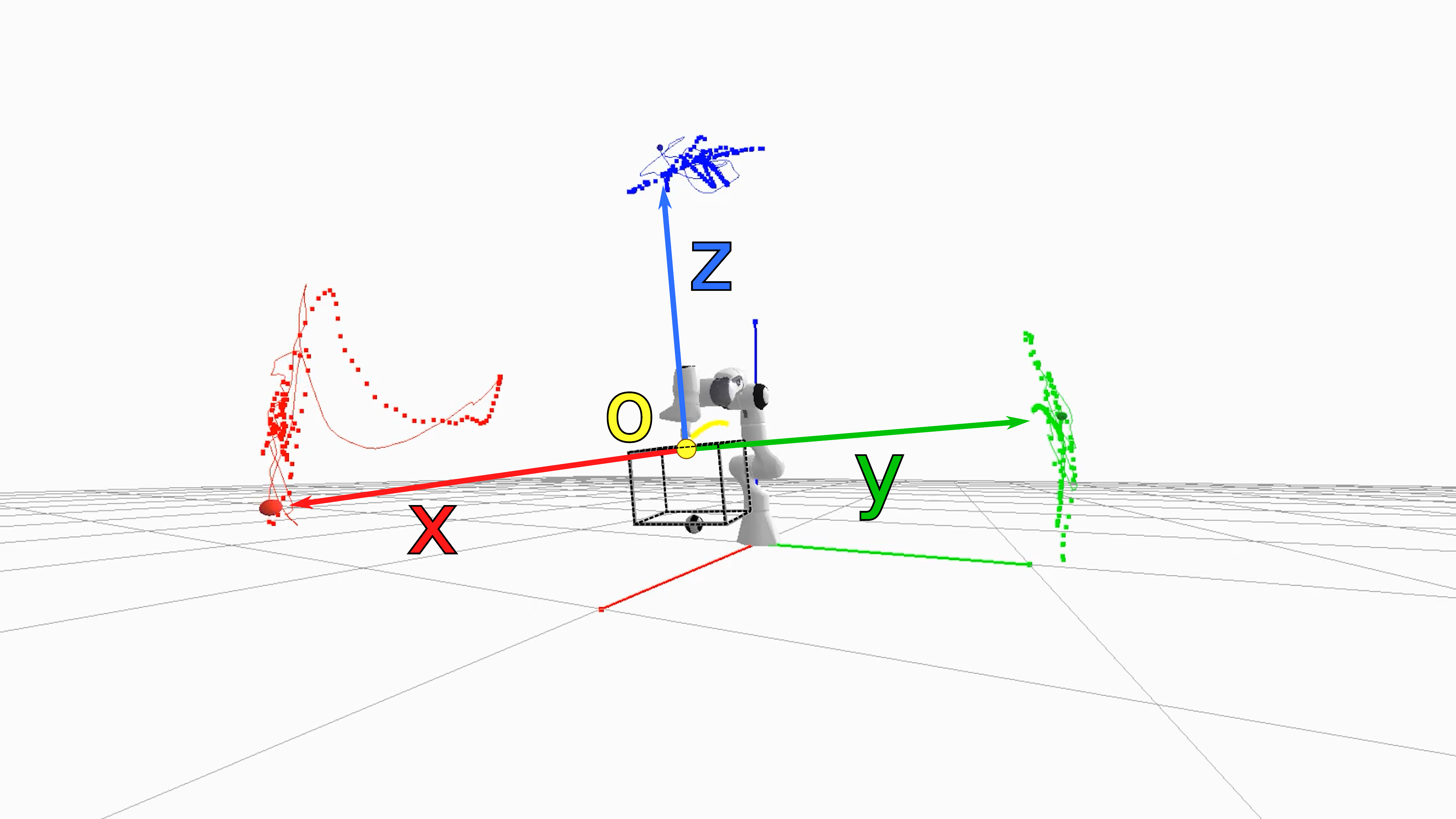Figure 8. Rendering of the simulation for the underactuated mechanism from Section 2.5, where the four reference points for the origin, unit x, y, and z vectors are shown. The trace of the simulated trajectory is visualized by the solid lines, the ground-truth trajectories of the markers are shown as dotted lines.

We first identify the simulation parameters pertaining to the inertial properties of the box and the friction parameters of the universal joint. As shown in Table 3 (a), the symmetric inertia matrix of the box is fully determined by the first six parameters, followed by the 3D center of mass. We have measured the mass to be 920g, so we do not need to estimate it. We simulate the universal joint with velocity-dependent damping, with possibly different friction coefficients for both degrees of freedom. The simulation parameters yielding the most accurate fit to a ground-truth trajectory from the physical robot shaking an empty box is shown in Figure 9. We were able to find such parameters via SVGD, CSVGD and Emcee (shown is a parameter configuration from the particle distribution estimated by CSVGD with the highest likelihood).

While the simulated trajectory matches the real data significantly better after the inertial parameters of the empty box have been identified (Figure 9 (b)) than before (Figure (a)), a reality gap remains. We believe this to be a result from a slight modeling error that the rigid body simulator cannot capture, e.g. the top of the box where the universal joint is attached bends slightly while the box is moving, and there may be slight geometric offsets between the real system and the model of it we use in the simulator. The latter parameters could have been further identified with our approach, nonetheless the simulation given the identified parameters is sufficient to be used in the next phase of the inference experiment.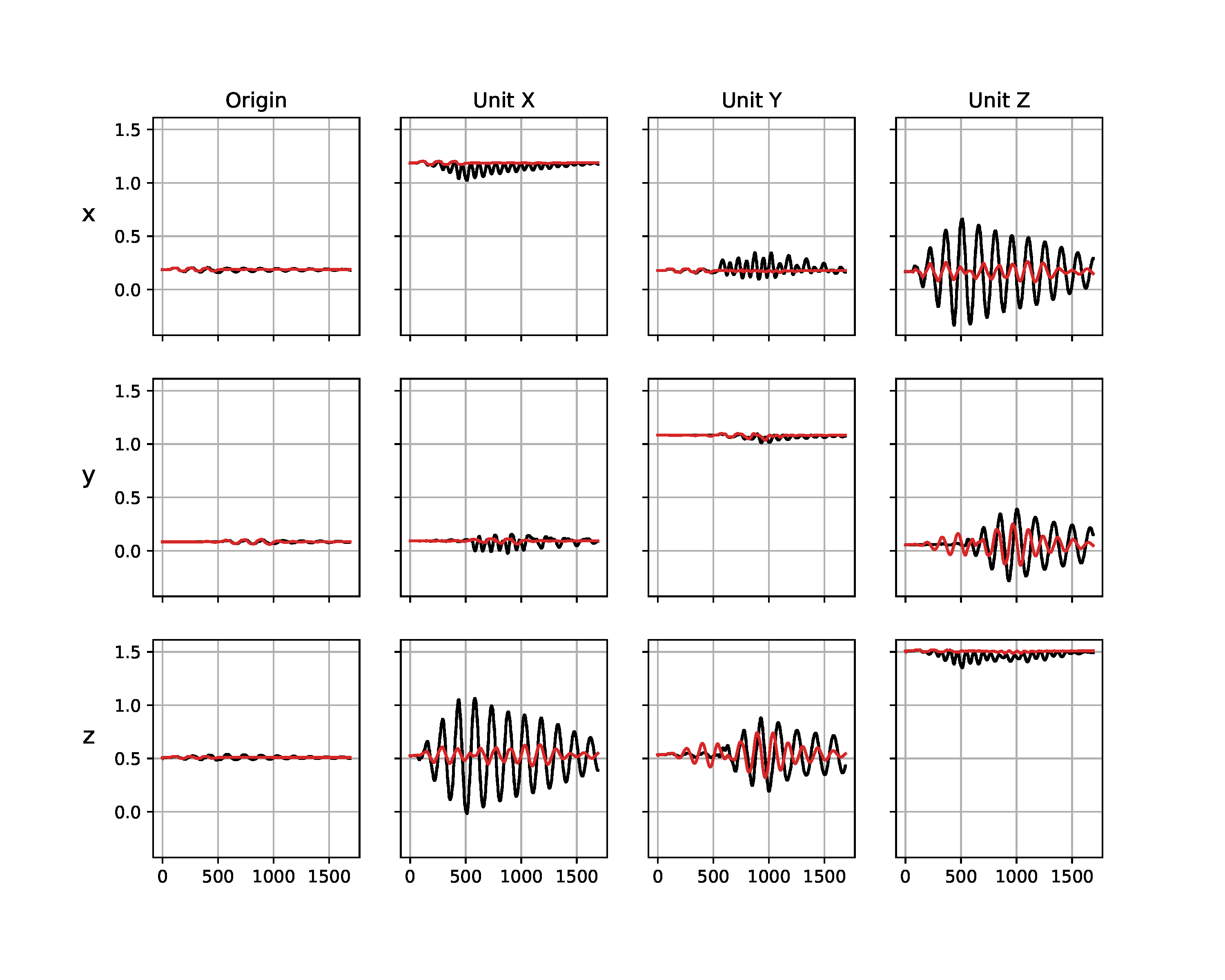(a) Before identification of empty box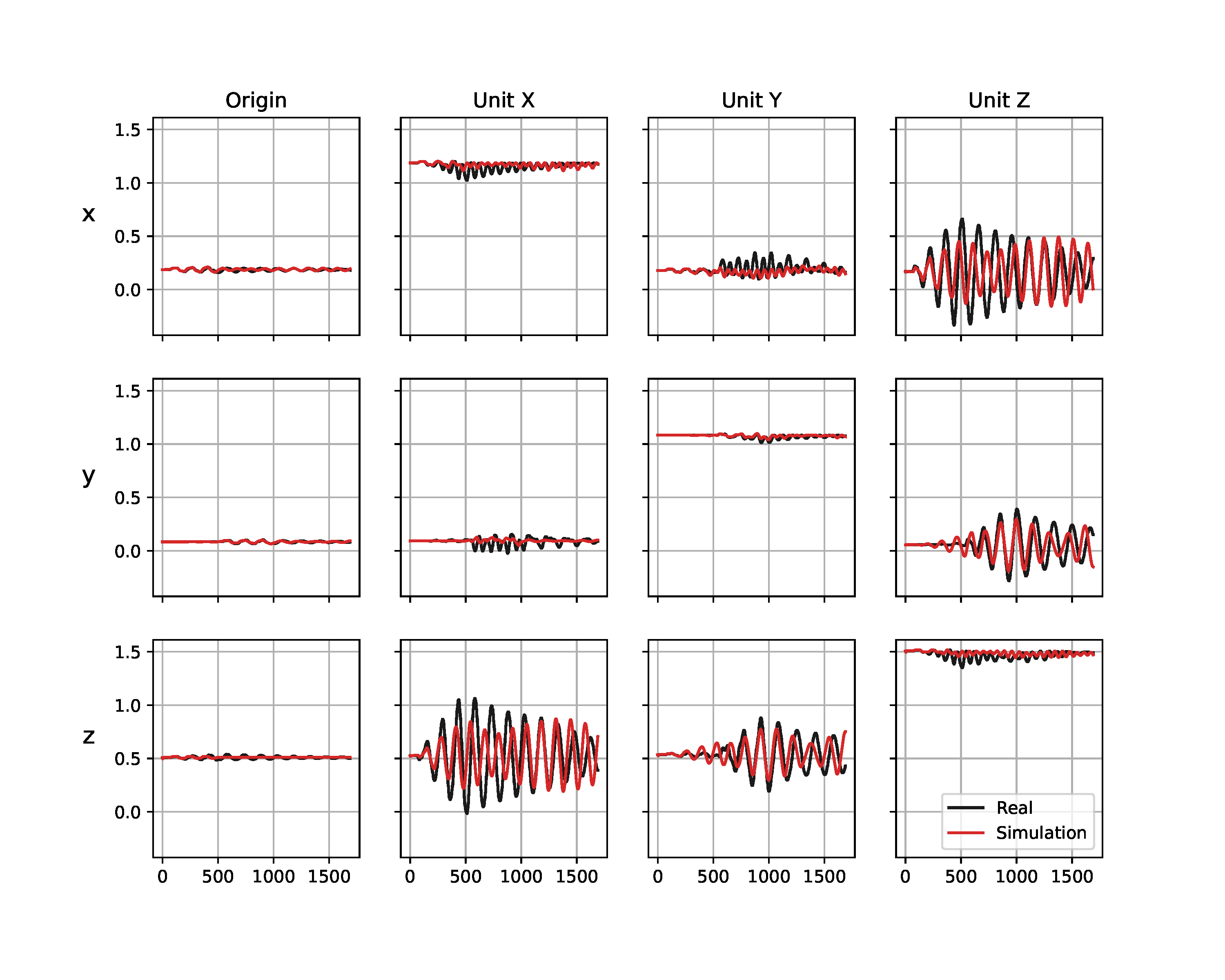(b) After identification of empty box

Figure 9. Trajectories from the Panda robot arm shaking an empty box. Visualized are the simulated (red) and real (black) observations before (a) and after (b) the inertial parameters of the empty box and the friction from the universal joint (Table 3 (a)) have been identified. The columns correspond to the four reference points in the frame of the box (see a rendering of them in Figure 8), the rows show the $x$, $y$, and $z$ axes of these reference points in meters. The horizontal axes show the time step.

Given the parameters found in the first phase, we now investigate how well the various approaches can cope with dependent variables. By fixing two 500g to the bottom of the acrylic box, the 2D locations of such weights need to be inferred. Naturally, such assignment is symmetric, i.e. weight 1 and 2 can swap locations without affecting the dynamics. What would significantly alter the dynamics, however, is an unbalanced configuration of the weights which would cause the box to tilt.

Parameter Minimum Maximum
Box $I_{xx}$ 0.05 $\kg\cdot\meter^2$ 0.1 $\kg\cdot\meter^2$
$I_{yy}$ 0.05 $\kg\cdot\meter^2$ 0.1 $\kg\cdot\meter^2$
$I_{zz}$ 0.05 $\kg\cdot\meter^2$ 0.1 $\kg\cdot\meter^2$
$I_{xy}$ -0.01 $\kg\cdot\meter^2$ 0.01 $\kg\cdot\meter^2$
$I_{xz}$ -0.01 $\kg\cdot\meter^2$ 0.01 $\kg\cdot\meter^2$
$I_{yz}$ -0.01 $\kg\cdot\meter^2$ 0.01 $\kg\cdot\meter^2$
COM $x$ -0.005 $\meter$ 0.005 $\meter$
COM $y$ -0.005 $\meter$ 0.005 $\meter$
COM $z$ 0.1 $\meter$ 0.4 $\meter$
U-Joint Friction DOF 1 0.0 0.15
Friction DOF 2 0.0 0.15

(a) Phase I

Parameter Minimum Maximum
Weight 1 Position $x$ -0.14 $\meter$ 0.14 $\meter$
Position $y$ -0.08 $\meter$ 0.08 $\meter$
Weight 2 Position $x$ -0.14 $\meter$ 0.14 $\meter$
Position $y$ -0.08 $\meter$ 0.08 $\meter$

(b) Phase II

Table 3. Parameters to be estimated and their ranges for the two estimation phases of the underactuated mechanism experiment from Section 2.5.

We use 50 particles and run each estimation algorithm for 500 iterations. For each baseline method, we carefully tuned the hyper parameters to facilitate a fair comparison. Such tuning included selecting an appropriate measurement noise variance, which, as we observed on Emcee and SGLD in particular, had a significant influence on the exploration behavior of these algorithms. With a larger observation noise variance the resulting posterior distribution became wider, however we were unable to attain such behavior with the NUTS estimator whose iterates quickly collapsed to a single point at the center of the box (see Figure 10 (d)). Similarly, CEM immediately became stuck in the suboptimal configuration shown in Figure 10 (b). Nonetheless, after 500 iterations, all methods predicted weight positions that were aligned opposite to one another to balance the box.

As can be seen in Figure 10 (e), SVGD achieves a fairly predictive posterior approximation, with many particles aligned close to the true vertical position at $y=0$. With the introduction of the multiple-shooting constraints, Constrained SVGD (CSVGD) converges significantly faster to a posterior distribution that accurately captures the true locations of the box, while retaining the exploration performance of SVGD that spreads out the particles over multiple modes, as shown in Figure 10 (f).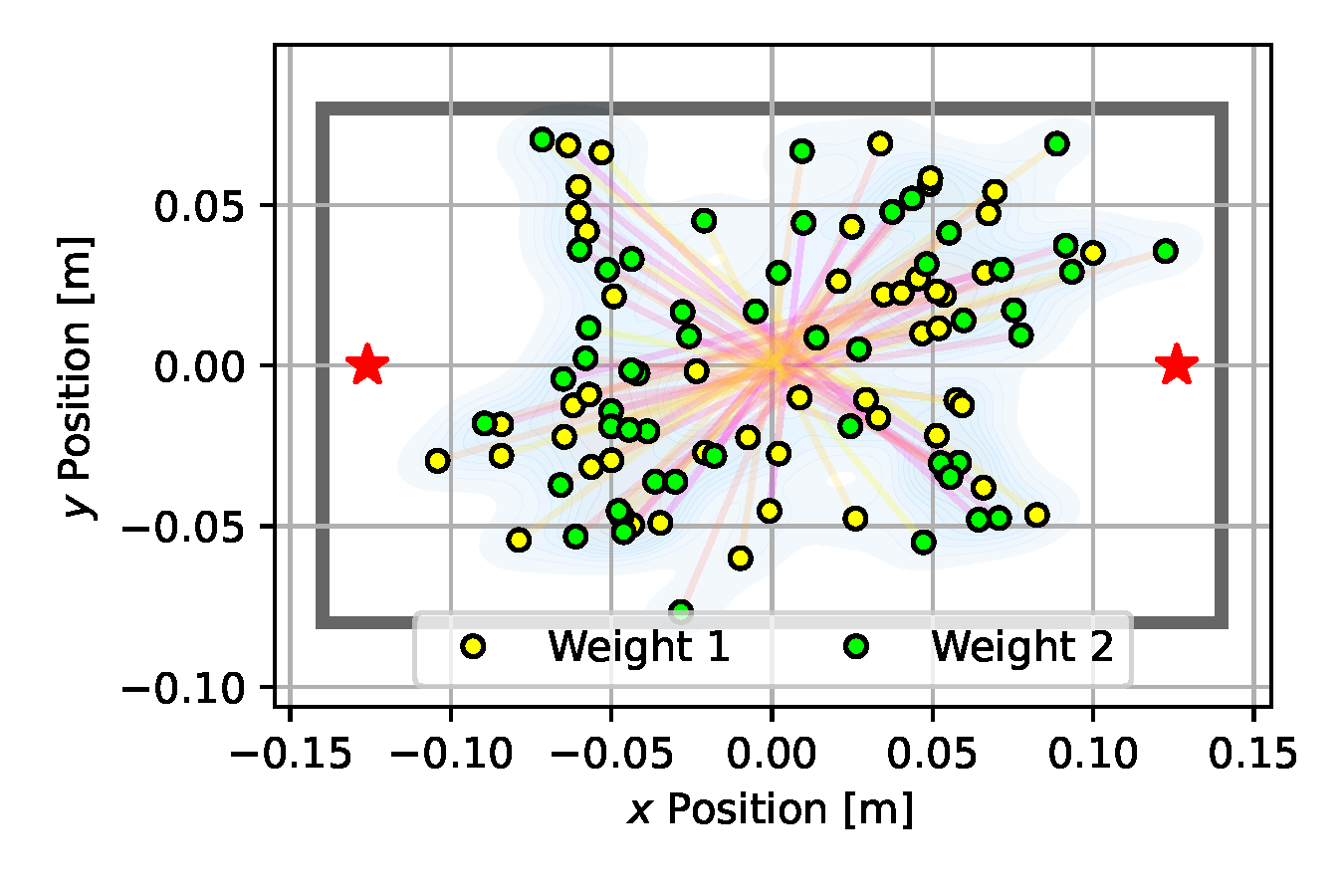(a) Emcee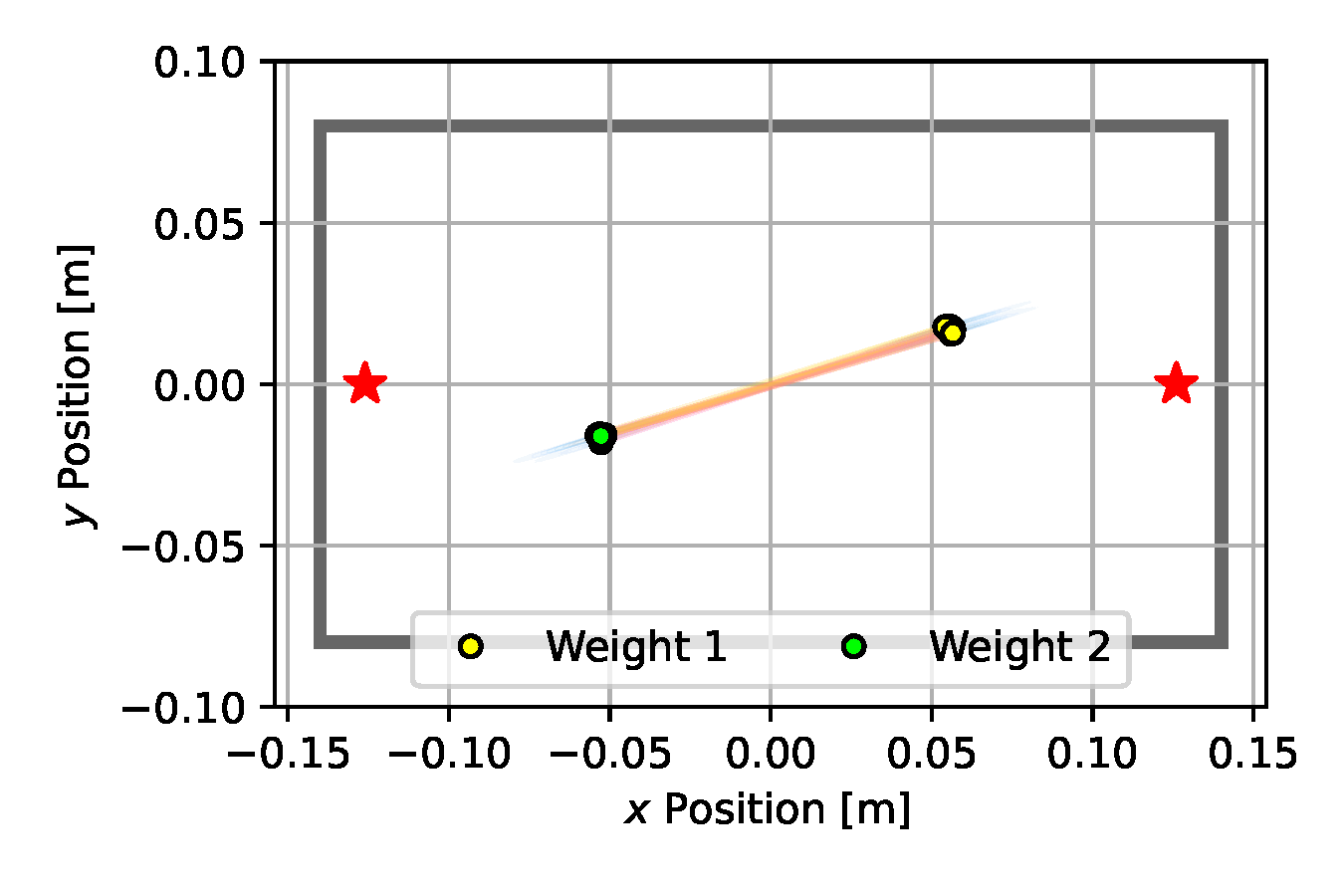(b) CEM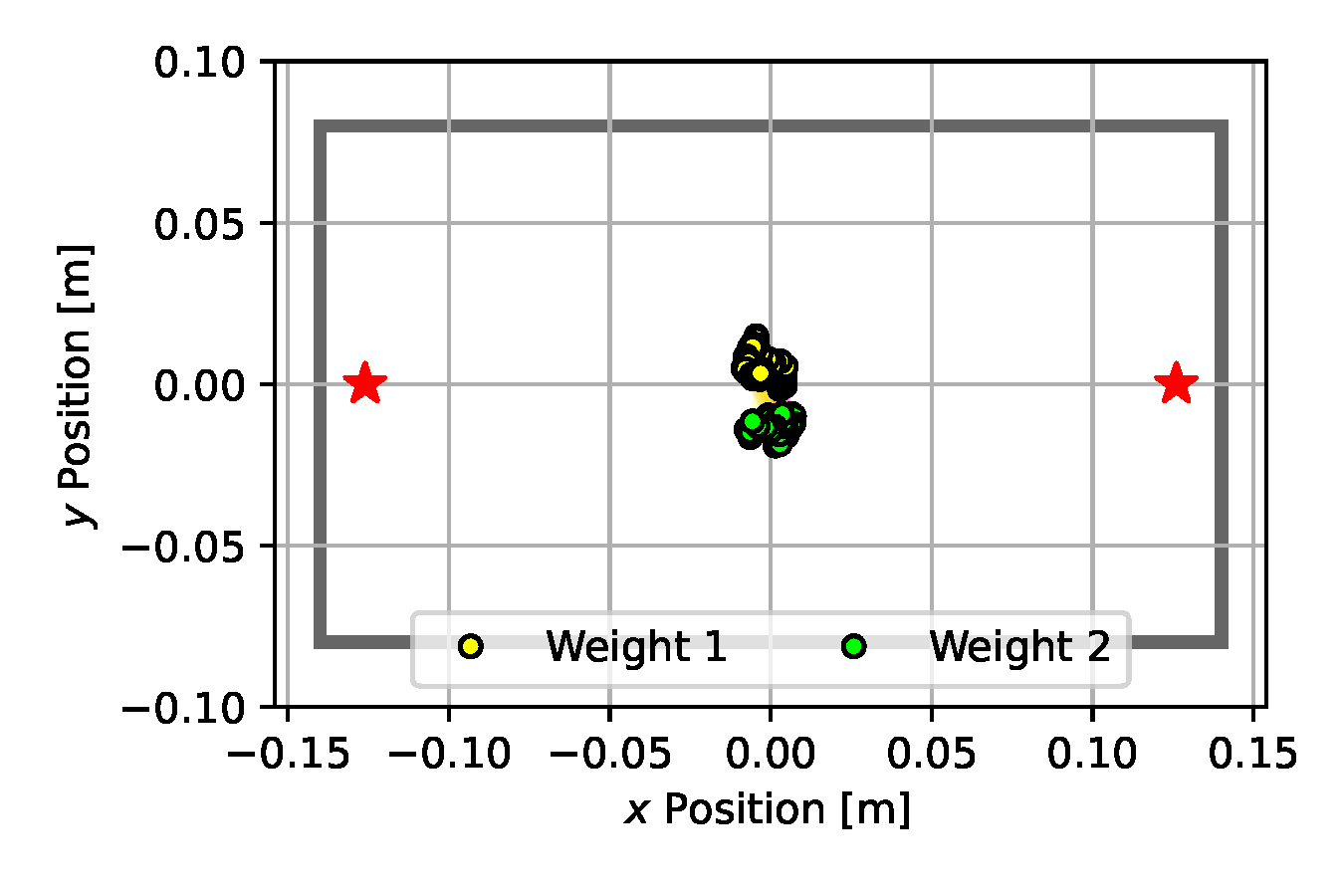(c) SGLD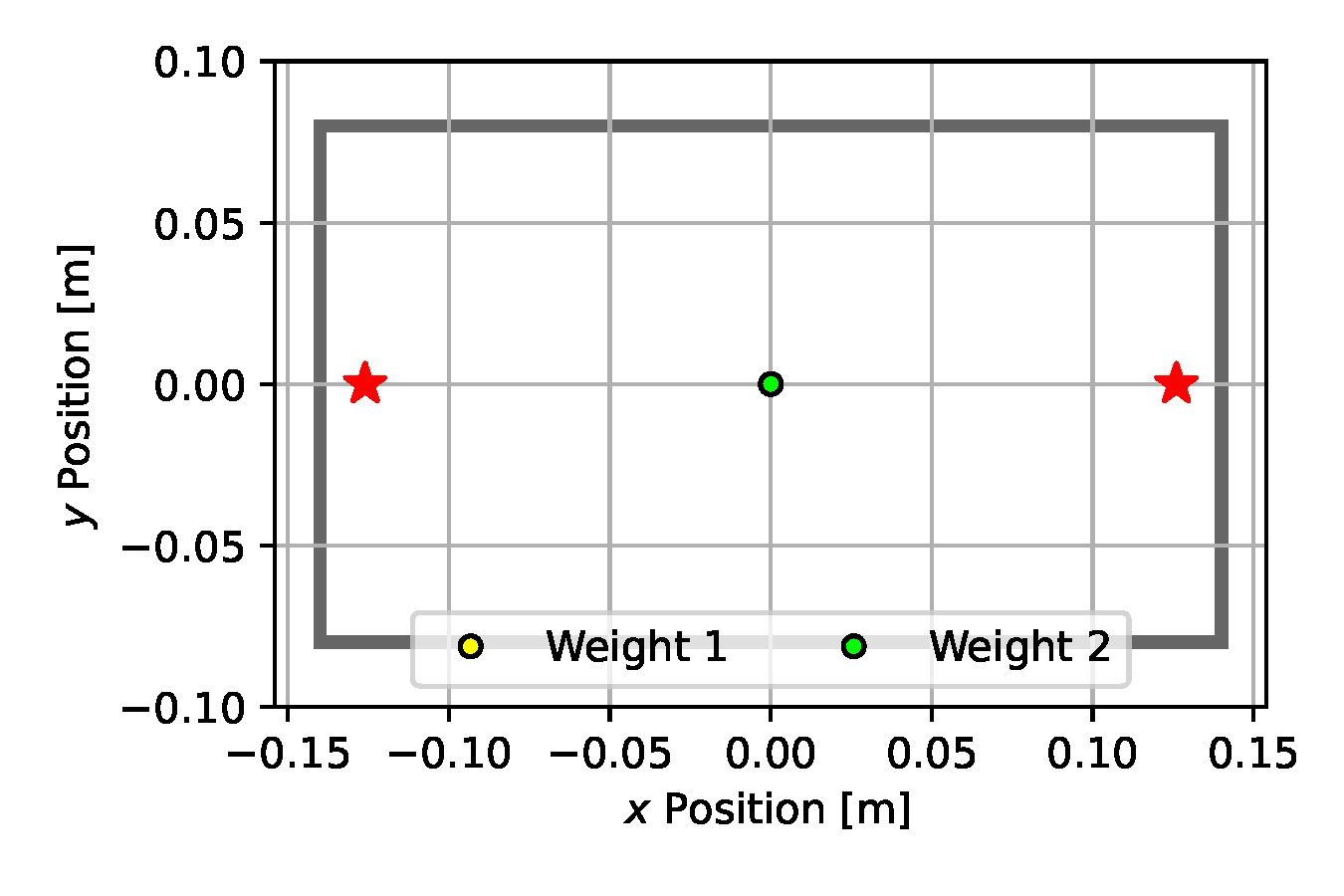(d) NUTS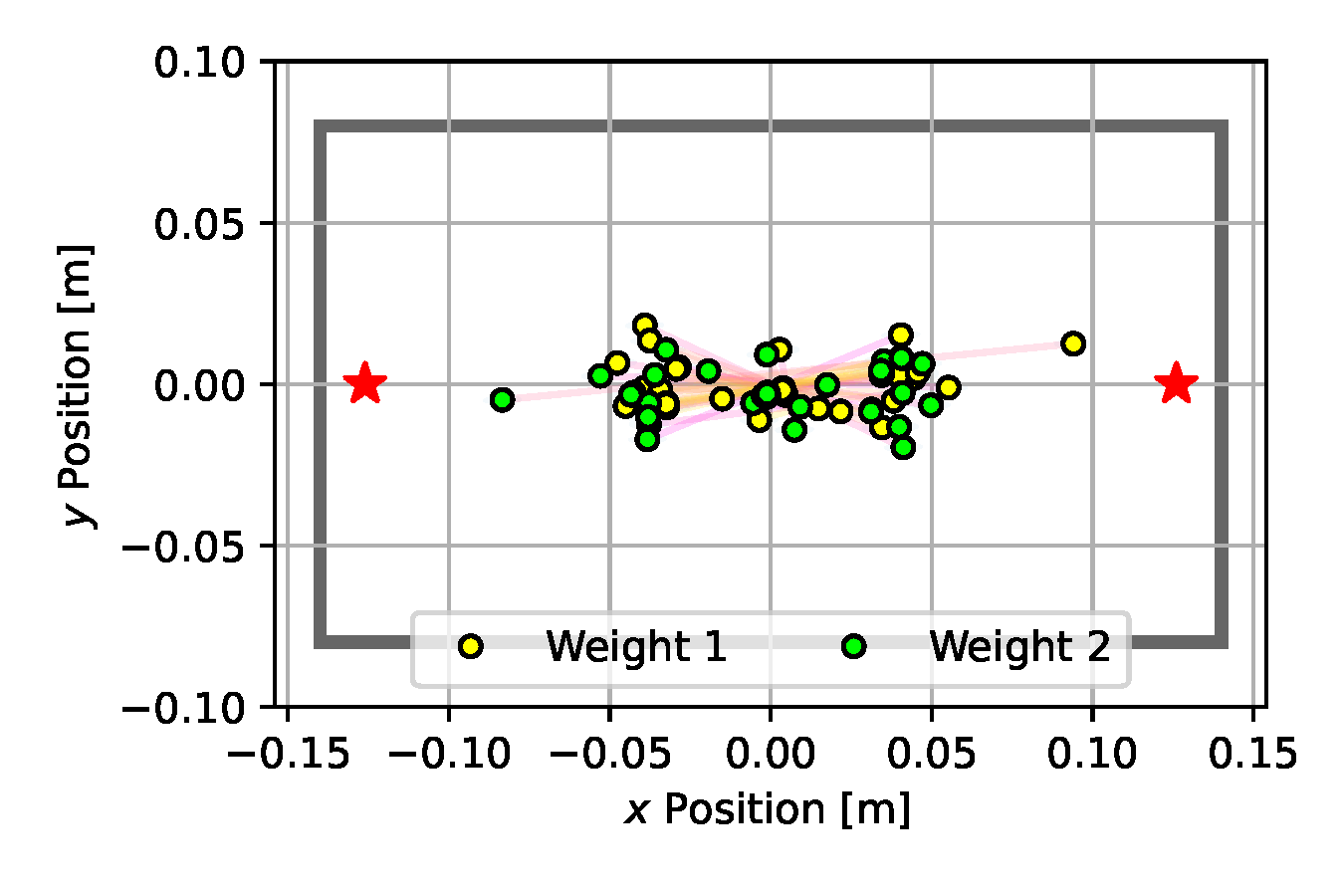(e) SVGD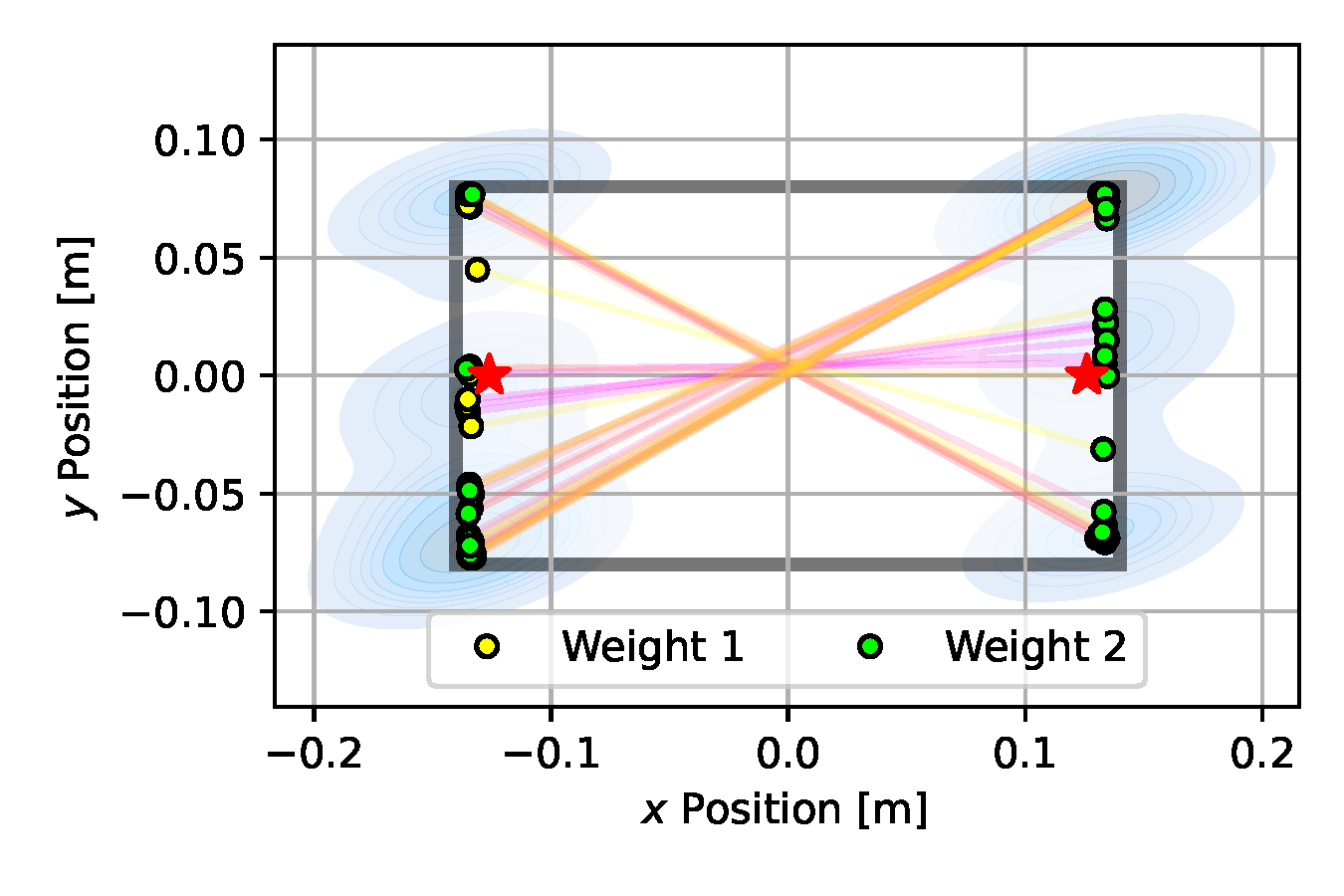(f) CSVGD

Figure 10. Posterior plots over the 2D weight locations approximated by the estimation algorithms applied to the underactuated mechanism experiment from Section 2.5. Blue shades indicate a Gaussian kernel density estimation computed over the inferred parameter samples. Since it is an unbounded kernel density estimate, the blue shades cross the parameter boundaries in certain areas (e.g. for CSVGD), while in reality none of the estimated particles violate the parameter limits.

### References

• J. Burkardt. Sobol - the sobol quasirandom sequence. https://people.sc.fsu.edu/~jburkardt/cpp_src/sobol/sobol.html. Accessed: 2021-06-23.
• B. L. Fox. Algorithm 647: Implementation and relative efficiency of quasirandom sequence generators. ACM Trans. Math. Softw., 12(4):362–376, Dec. 1986. ISSN 0098-3500. doi:10.1145/22721.356187.
• R. Chou, Y. Boers, M. Podt, and M. Geist. Performance evaluation for particle filters. In 14th International Conference on Information Fusion, pages 1–7, July 2011.
• Q. Wang, S. R. Kulkarni, and S. Verdu. Divergence Estimation for Multidimensional Densities Via $k$-Nearest-Neighbor Distances. IEEE Transactions on Information Theory, 55(5):2392–2405, May 2009. ISSN 0018-9448. doi:10.1109/TIT.2009.2016060.
• E. Heiden, D. Millard, E. Coumans, Y. Sheng, and G. S. Sukhatme. NeuralSim: Augmenting differentiable simulators with neural networks. In Proceedings of the IEEE International Conference on Robotics and Automation (ICRA), 2021. URL https://github.com/google-research/tiny-differentiable-simulator.
• R. Featherstone. Rigid Body Dynamics Algorithms. Springer-Verlag, Berlin, Heidelberg, 2007. ISBN 0387743146.
• L. S. Pontryagin. Mathematical theory of optimal processes. Routledge, 2018.
• A. Asseman, T. Kornuta, and A. Ozcan. Learning beyond simulated physics. In Modeling and Decision-making in the Spatiotemporal Domain Workshop, 2018. URL https://openreview.net/forum?id=HylajWsRF7.
• F. Ramos, R. Possas, and D. Fox. BayesSim: Adaptive domain randomization via probabilistic inference for robotics simulators. In Proceedings of Robotics: Science and Systems, Freiburg im Breisgau, Germany, June 2019. doi:10.15607/RSS.2019.XV.029.
• C. M. Bishop. Mixture density networks. 1994.
• P. Kidger and T. Lyons. Signatory: differentiable computations of the signature and logsignature transforms, on both CPU and GPU. In International Conference on Learning Representations, 2021. https://github.com/patrick-kidger/signatory.
• C. E. Rasmussen and C. K. I. Williams. Gaussian Processes for Machine Learning (Adaptive Computation and Machine Learning). The MIT Press, 2005. ISBN 026218253X.
• T. Coleman David. Reducing the Barrier to Entry of Complex Robotic Software: a MoveIt! Case Study. 2014. doi:10.6092/JOSER_2014_05_01_P3. URL https://aisberg.unibg.it/handle/10446/87657. Publisher: Universita degli studi di Bergamo.
• D. Q. Huynh. Metrics for 3d rotations: Comparison and analysis. Journal of Mathematical Imaging and Vision, 35(2):155–164, 2009.

## Acknowledgements

This work was supported by a Google PhD Fellowship and a NASA Space Technology Research Fellowship, grant number 80NSSC19K1182.

G.S. Sukhatme holds concurrent appointments as a Professor at USC and as an Amazon Scholar. This paper describes work performed at USC and is not associated with Amazon.

Last updated on September 20, 2021